Jekyll2023-11-28T18:35:29+08:00https://blog.42yeah.is/feed.xmlOf Shaders &amp; TrianglesA semi-curated blog of computer graphics and rendering.Of Shaders &amp; Triangles: Blog Update2023-10-07T22:36:30+08:002023-10-07T22:36:30+08:00https://blog.42yeah.is/meta/2023/10/07/blog-updateHey guys, it’s been a while. Since I have made up my mind to submit a new blog post every Saturday this year, I have since posted exactly 42 blog posts. It’s not the number I have intended to reach (in fact, I didn’t think I’d make it past 5~6 weeks); and it feels magical. But from now on, this blog will no longer receive reguar updates. The reason? I have landed on a job. It’s the first computer graphics job I’ve ever gotten, and although it’s more frontend-y, so to speak, it also fullfills my desire to work in computer graphics-related fields. As I can’t really balance between my graduation paper, the new job, and my passion baby project, I suppose one of them have to go.

Looking back, we have set sail on so many different journeys. Every week is a different adventure. We took a sneak peak at the highs and lows, the basics and advanced topics of computer graphics (and something else): from framebuffers to raymarching, from dithering to ReSTIR. Alright, sometimes I got lazy, and I just reuse the old ones - but hey, it counts anyway.

To all my subscribed readers, friends, and random internet strangers: thank you for reading my blog. I know, it didn’t reach one year, and I am kinda sad about that - but nevertheless, I am honored to have you here. And worry not - I didn’t say I will stop updating it entirely, just that it will no longer receive regular updates. Every once in a while, when the strong urge to share arises again, this blog shall always receive new blog posts. Maybe I will even share new things encountered while I was working my job. And until then, I will see you all later.

]]>
Installing PyTorch Geometric w.r.t. CUDA Version2023-09-30T22:08:30+08:002023-09-30T22:08:30+08:00https://blog.42yeah.is/cuda/2023/09/30/torch-geometricI’ve been fiddling with PyTorch Geometric installation lately, and I believe we all know that although being an awesome library, sometimes it can be notoriously hard to get it to work in the first place. Especially if your CUDA version is way too low. That can be very, very problematic. So, let’s assume we have an unlisted CUDA version (11.1 in my case,) and let’s try to make it work!

## Installing PyTorch

So the very first thing is to install PyTorch. And to install PyTorch (the GPU-enabled version, obviously,) you can follow this helpful link on the official website. If, however, our CUDA version is too low and becomes unlisted (again,) we can follow this link and just search for our version. In our case (CUDA 11.1,) we see that the latest supported version is torch 1.10.1.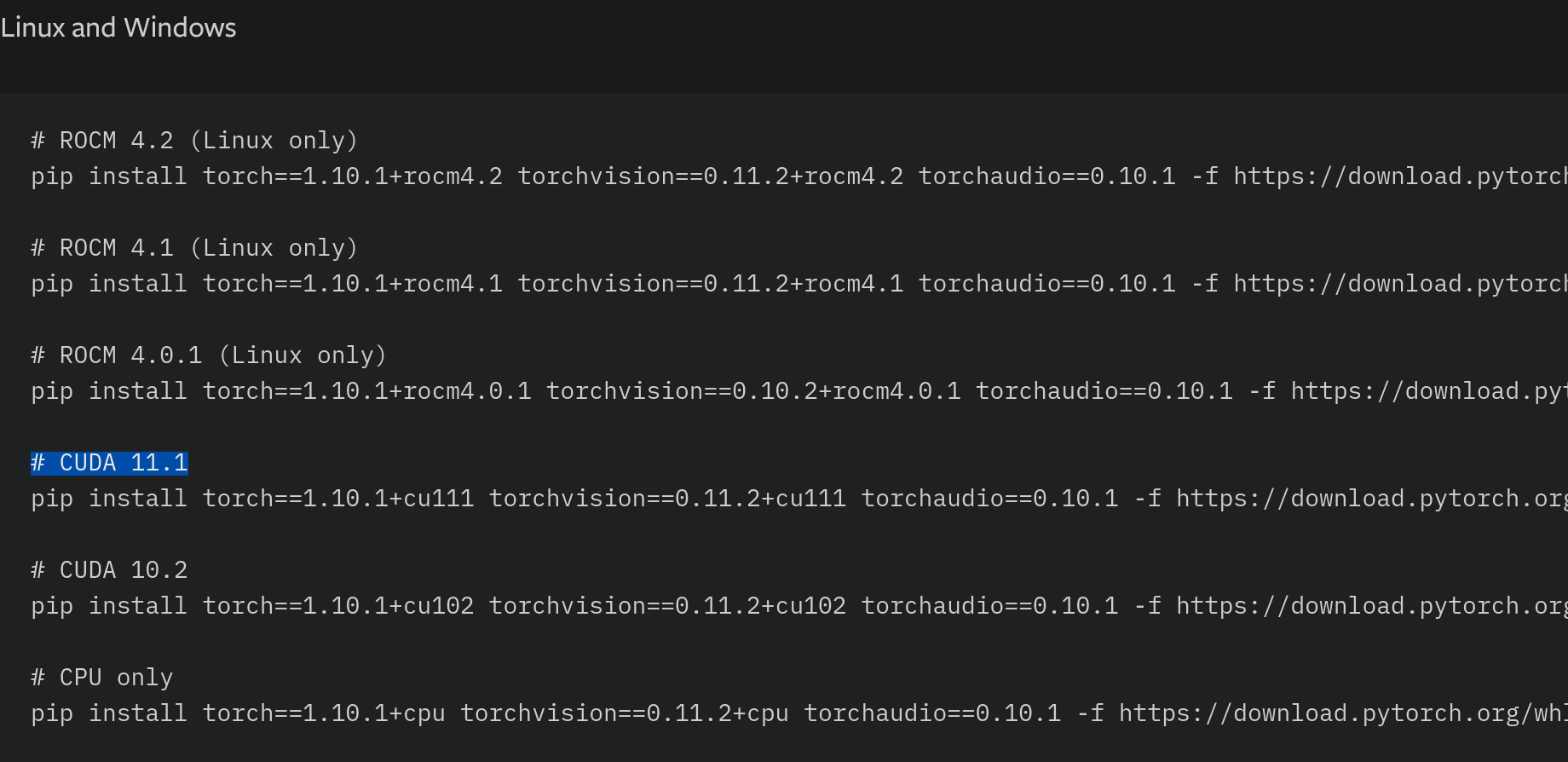So we copy & paste that into our command line and BAM! PyTorch done.

After that, try to import torch and verify that we can indeed create variables using cuda:

import torch
torch.randn(1, 1, device='cuda')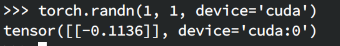Wow! It is on device! Let’s go!

For unsupported OSes, sometimes there is no precompiled PyTorch wheels, and that calls for a complete compilation from source. If such cases arise, it is very important for us to choose one compiler throughout the entire compilation (i.e. the compiler needs to be the same for both PyTorch and PyTorch Geometric.) If you are using an archaic version of, say, Ubuntu, which leads to an ancient GCC and G++, I highly recommend you to install Clang instead. For Ubuntu users, you can install Clang using llvm.sh, with a comprehensive guide available here. The Clang version can’t be too new or too old, otherwise we can’t compile PyTorch Geometric. My recommended version is clang-6.0, which works for me.

We can tell pip to use our new compiler once we have finished the installation:

export CC=clang-6.0
export CXX=clang++-6.0

# And now install PyTorch
pip install torch==...


## Installing PyTorch Geometric

PyTorch Geometric (PyG for short) is more like a toolkit with four major dependencies:

• torch_scatter
• torch_sparse
• torch_cluster
• torch_spline_conv
• pyg_lib (???)

If you have relatively new PyTorch and CUDA installed, you can just follow the guide from its official website. Otherwise, we will have to take the matter into our own hands. Note that on its official website, there is a find link for specific PyTorch and CUDA versions: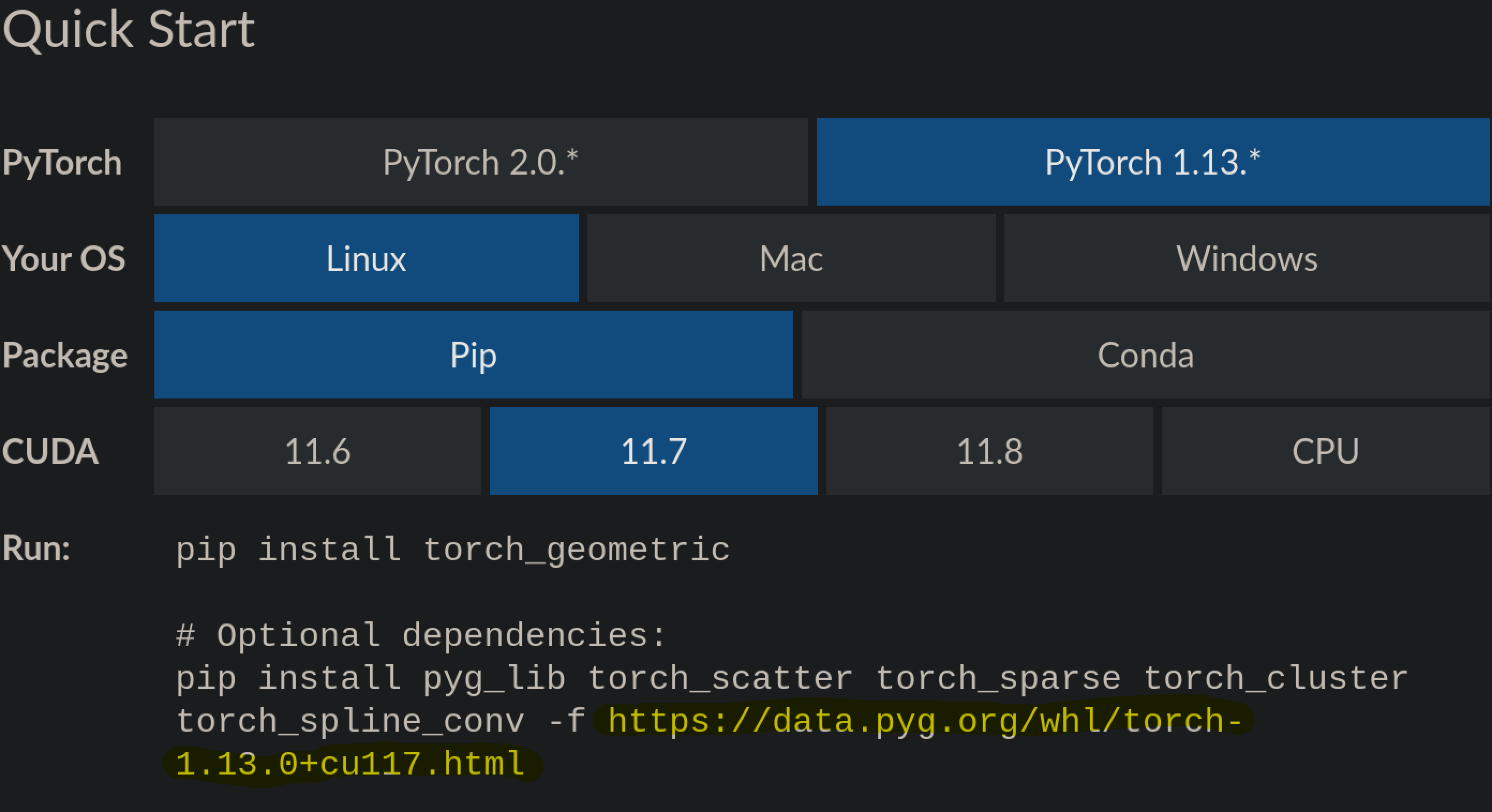By copy-pasting it and replacing the torch version and CUDA version with our version (PyTorch 1.10.1 + CUDA 11.1), we get https://data.pyg.org/whl/torch-1.10.1+cu111.html. And after accessing it we are presented with a list of supported versions of PyG dependencies: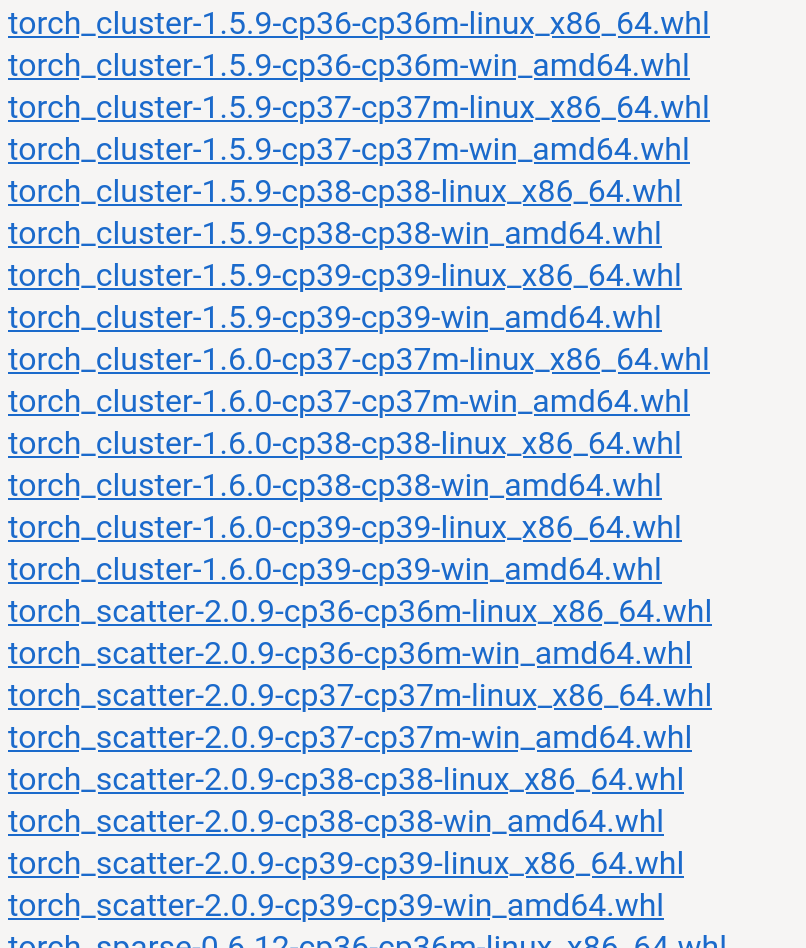After a quick look we can see that supported versions of PyG dependencies given our CUDA and PyTorch versions are:

• torch_cluster: 1.5.9, 1.6.0
• torch_scatter: 2.0.9
• torch_sparse: 0.6.12, 0.6.13
• torch_spline_conv: 1.2.1

Now we simply need to pick their newest versions (or not as new, it’s really up to you) and pip install them.

pip install torch_scatter==2.0.9 torch_sparse==0.6.13 torch_cluster==1.6.0 torch_spline_conv==1.2.1 -f https://data.pyg.org/whl/torch-1.10.1+cu111.html
pip install torch_geometric


WARNING! It’s very important that you specify the version here, otherwise pip will completely disregard the find link and just download the newest version of the four dependencies. You might have noticed that pyg_lib is absent from the above list. I have noticed that as well, but since my task then was to simply install PyG, I disregarded the discrepency and no errors comes off it. I guess my PyTorch Geometric version is too old for the pyg_lib thing.

If you want some new thrilling adventure (or if your OS is too old, as stated above,) you can also compile PyG from source. The procedure is basically the same as above. We have to guarantee the compiler remains the same.

However, if you are using Clang as advised, you will encounter two errors.

1. The linker will complain something about “libomp5-10”. I am not sure about this, but this seems to be due to PyTorch’s wheel’s own OpenMP library clashes with system-wide OpenMP. This can be solved by specifying the OpenMP library: CFLAGS="-fopenmp=libiomp5" pip install torch_cluster --no-cache
2. Some weird template error at PyTorch’s variant.h. Funnily enough, I found the solution to both issues on macOS devices with Clang trying to install PyG. This reply solves this issue.

After a long wait, you should be able to build all four (five?) dependencies from source. After that, just install PyG (or build it as well.)

## Conclusion

That’s it! A grand adventure of version wrangling, all due to the outdated CUDA version on the server side. But I guess that’s why it’s fun, right? To verify that PyG has been definitively successfully installed, I try to import all four dependencies independently, and try to print out its CUDA version:

import torch_scatter
print(torch_scatter.torch.version.cuda)

# ... and so on.


And that’s about it. Have fun using PyG!

]]>
Worley and His Noise (Worley Noise/Voronoi Noise)2023-09-23T22:14:30+08:002023-09-23T22:14:30+08:00https://blog.42yeah.is/rendering/noise/2023/09/23/voronoiThis is a blog post from the past. Originally written at 2020-02-11.

## Introduction

Now, you must be wondering why you should read my blog post if there are so many far better tutorials out there. And you are right, boi. I have absolutely no confidence in what I write, and that’s why I leave all those references. So, Worley Noise! If you didn’t hear about this before, or Cellular Noise, or something to that extent, maybe you’ve heard the Voronoi diagram, right? It looks a little bit like this:And with a little bit of tweak, BAM! It becomes Worley Noise! Let’s begin!

## Implementation

It’s actually really, really simple. First, Let’s get a canvas: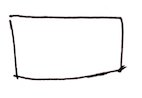And then add a few random dots: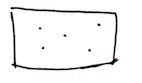Then, for every pixel inside this canvas, we calculate the closest dot to the pixel. After this iteration, every pixel will be colored (because there will always be a closest point), and things would look triangular and cool.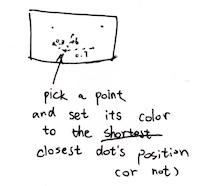Well, doodling sucks. Let’s take a look a the code!

// Five random points
vec2 points;
points = vec2(0.3, 0.8);
points = vec2(1.2, 0.1);
points = vec2(1.0, 0.5);
points = vec2(0.2, 0.4);
points = vec2(0.6, 1.0);

// Keep track of the minimum distance,
float m = 1.0;
// and the closest dot
vec2 closestDot;
for (int i = 0; i < 5; i++) {
float dist = distance(uv, points[i]);
// Distance closer than minimum distance?
if (dist < m) {
// Update it
m = dist;
closestDot = points[i];
}
}

// Set the pixel output color's R & G component to be the position of the dot
gl_FragColor = vec4(closestDot, 0.0, 1.0);


And now obviously all color that is closest to the point would be colored as areas. Which is very cool! And yeah, it is that easy. It’s cool, because it gets irregular shapes.

### No ifs, no buts

Coloring areas are nice and all, but it’s a little bit boring. Also it gets this if. We all know that ifs in GLSL is bad! Bad for performance! Bad if! Well, we can take a step back, and instead of coloring areas, we set the output pixel’s color to the closest point’s distance. In this way, our output image would become a continuous grayscale (or whatever scale you prefer) image: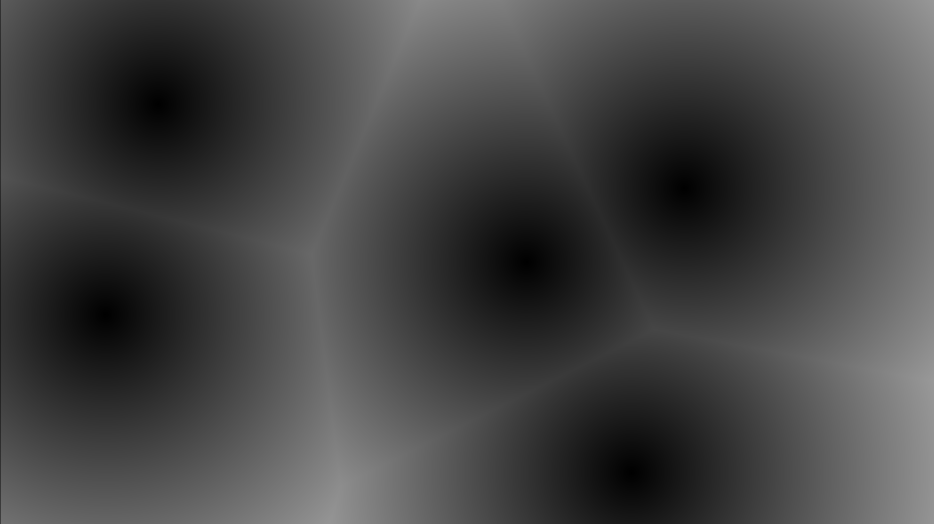This, by the way, could make really good looking lava moats, or dry rocks, if you could think about a way to animate those random dots, which we will cover later:Just a different color! Well, let’s take a look at the source!

vec2 points;
points = vec2(0.3, 0.8);
points = vec2(1.2, 0.1);
points = vec2(1.0, 0.5);
points = vec2(0.2, 0.4);
points = vec2(0.6, 1.0);

// ONLY keep track of the minimum distance.
float m = 1.0;
for (int i = 0; i < 5; i++) {
float dist = distance(uv, points[i]);
m = min(m, dist); // Or just minimize it to
// m = min(m, distance(uv, points[i]));
}

// Set the pixel output color's grayscale component to be the distance to the closest point
gl_FragColor = vec4(m, m, m, 1.0);


Easy peasy lemon squeezy!

### Removing for

ifs are gone now, and that’s good; but for still exists. And as there is actually a if in for, for isn’t good and thus we should remove it as well. We could procedurally generate the points on the way. Not only it saves up memory as generating points use pure maths, we could have infinite points, and thus the voronoi diagram can expand to infinity.

But first, we will just take a look at how we will remove for. So how could we do that, actually? Well, of course we should use the space tiling technique:

uv *= 3.0; // zoom out; change it as you please
vec2 u = floor(uv);
vec2 f = fract(uv);
gl_FragColor = vec4(sin(u), 0.0, 1.0);


In this way, the space could be tiled elegantly: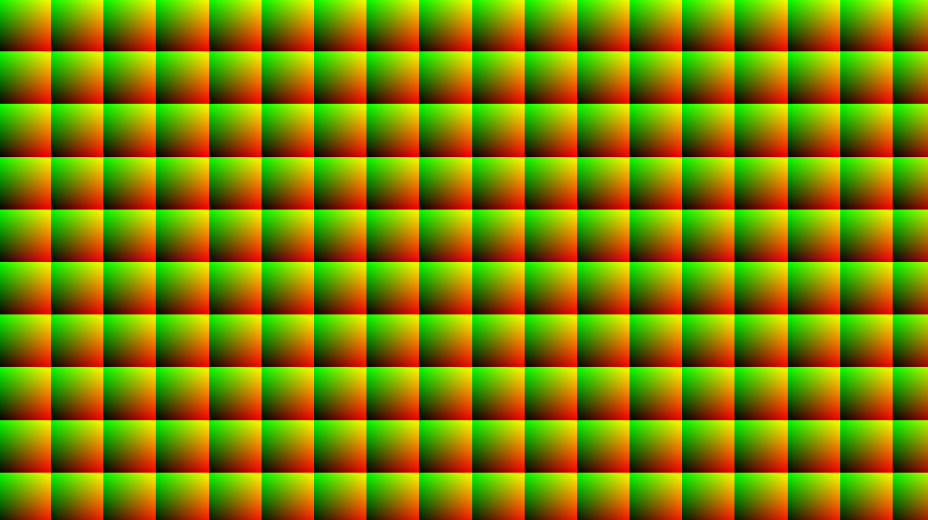every color grid is a tiled space. Also in this image, I zoomed way out so you could see the tiled effect properly. Now after tiling, f becomes our new uv; it is standarized, as it’s always in [(0, 0), (1, 1)). Then, We could just generate a point in every tile!

// our dear one-liner
vec2 rand(vec2 u) {
return fract(sin(vec2(dot(u,vec2(127.1, 311.7)),
dot(u,vec2(269.5, 183.3)))) * 43758.5453);
}

void main(void)
{
vec2 uv; // Get uv in some way

uv *= 3.0; // Zoom out
vec2 u = floor(uv);
vec2 f = fract(uv);
vec2 p = rand(u); // So every tile's point will always be the same

float m = distance(f, p);
gl_FragColor = vec4(
m, m, m,
1.0);
}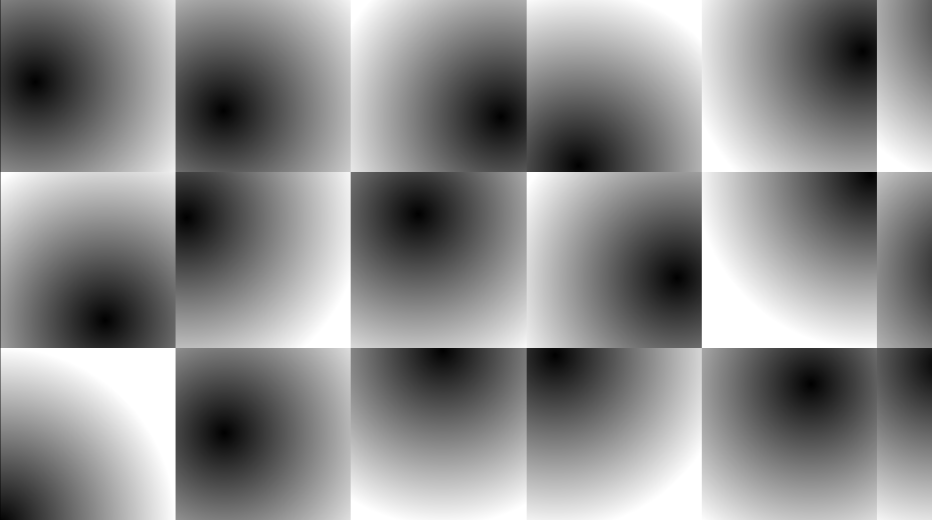Well, remember our rand function? If not, check it out here. After tiling & generating, we could make sure all tiles always get a point located at somewhere. However, it doesn’t look like the original Worley stuff again, because there is only one point left to compare; there is no way to get the position of other points!

### Or is there?

Well obviously there is! As we can see clearly, the closest point to a pixel only have at most 9 possibilities. Take the pixel in green circle for example.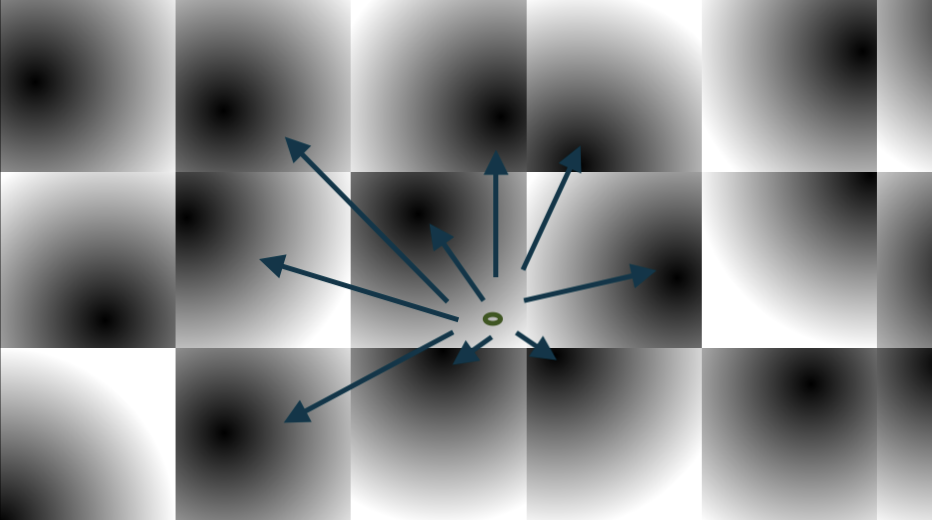In other words, its 9 neighbros. Other points are impossible to be closer to the 9 neighbors, right? So what we need now is a double loop (well, power comes at a cost):

vec2 rand(vec2 u) {
return fract(sin(vec2(dot(u,vec2(127.1, 311.7)),
dot(u,vec2(269.5, 183.3)))) * 43758.5453);
}

void main(void)
{
vec2 uv; // Go get it yourself

uv *= 3.0; // Zoom out
vec2 u = floor(uv);
vec2 f = fract(uv);
float p = 1.0; // Assume furthest
for (int y = -1; y <= 1; y++) {
for (int x = -1; x <= 1; x++) {
vec2 off = vec2(x, y);
p = min(p, distance(f - off, rand(u + off)));
}
}

gl_FragColor = vec4(
p, p, p,
1.0);
}


And here we go!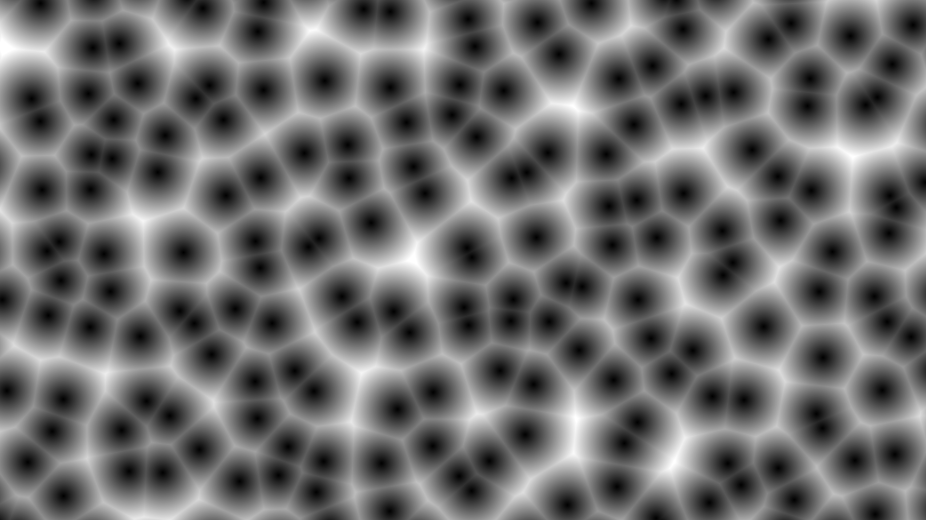*ZOOM*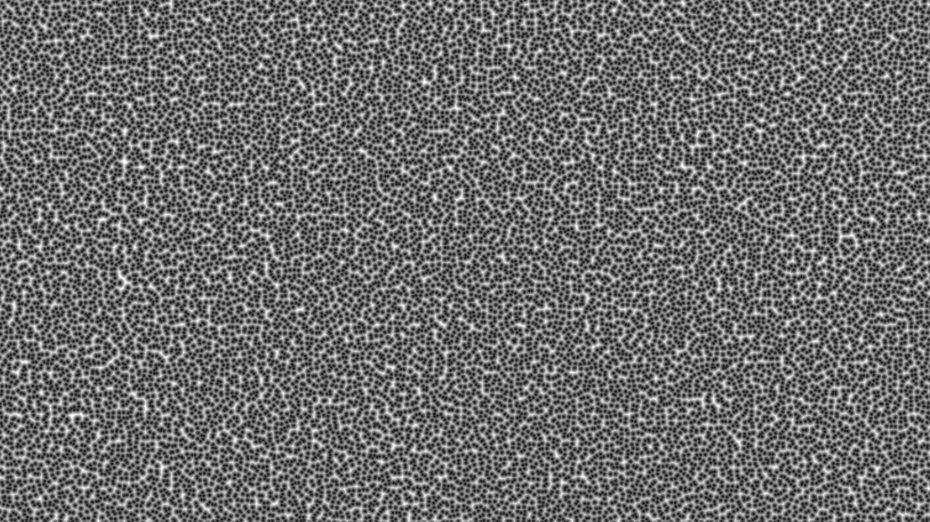Infinite Worley noise, at your disposal!

Adding isolines could make the thing kinda sorta looks like a triangular contour. First we are gonna use the Voronoi code, only it is the infinite version:

uv *= 10.0;

vec2 u = floor(uv);
vec2 f = fract(uv);
float m = 1.0;
vec2 mPos;
for (int y = -1; y <= 1; y++) {
for (int x = -1; x <= 1; x++) {
vec2 p = rand(u + vec2(x, y));
float d = distance(f - vec2(x, y), p);
if (d < m) {
m = d;
mPos = p;
}
}
}

vec3 color = vec3(rand(mPos), 1.0); // use rand so the color does not go out of bound
// and set B=1.0 so the screenshot looks samsungy

color -= abs(sin(m * 80.0)) * 0.01; // This line shows the isoline

gl_FragColor = vec4(
color,
1.0);


This is what it looks like without isoline: (Oooh, Samsung Galaxy something!)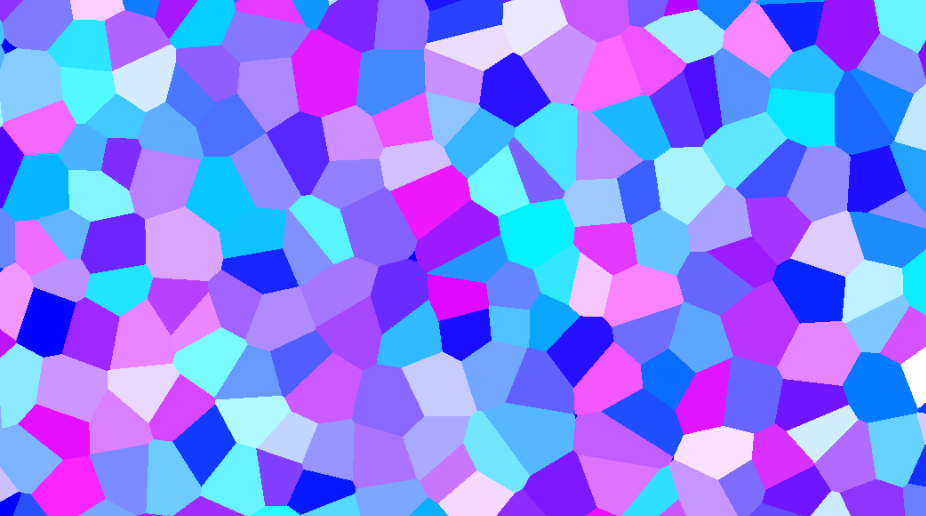Then we subtract the output color by abs(sin(distance to the point * n)) * k. tweak the n for the stripe count you want, and tweak k for the stripe obviousness. And when you set k = 1, and zoom level = 2.0 or something, you will get a cool looking neon effect! That’s exactly how I get the featured image. Also lower the zoom level. This is what it looks like with isoline: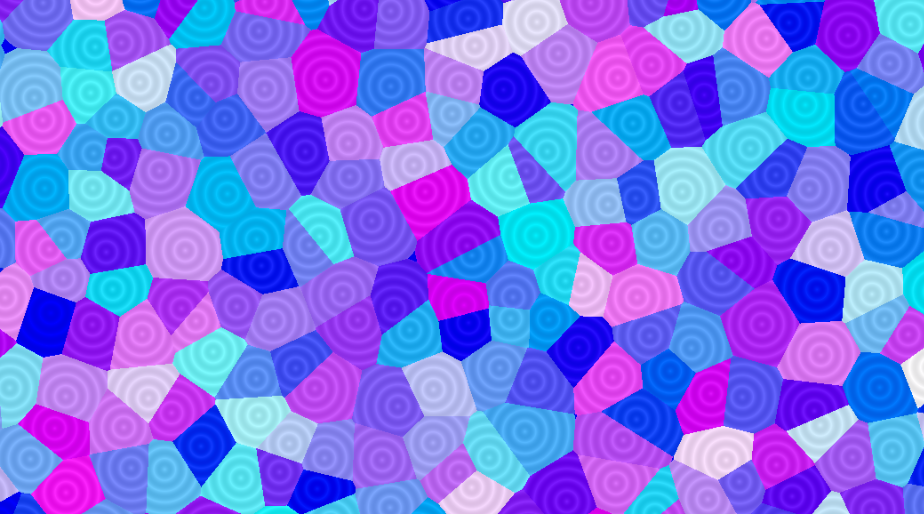### Animation

Animating the scene is extremely easy. Just use a sine function to move the generated points around (but not too much, otherwise it will move so far out it would actually be the closest in other tiles, and the image would look jagged). Also, the color hash trick above would not work, as the closest point will change now. It will result in rapidly blinking. Solution exists, of course; but you gotta think about a way.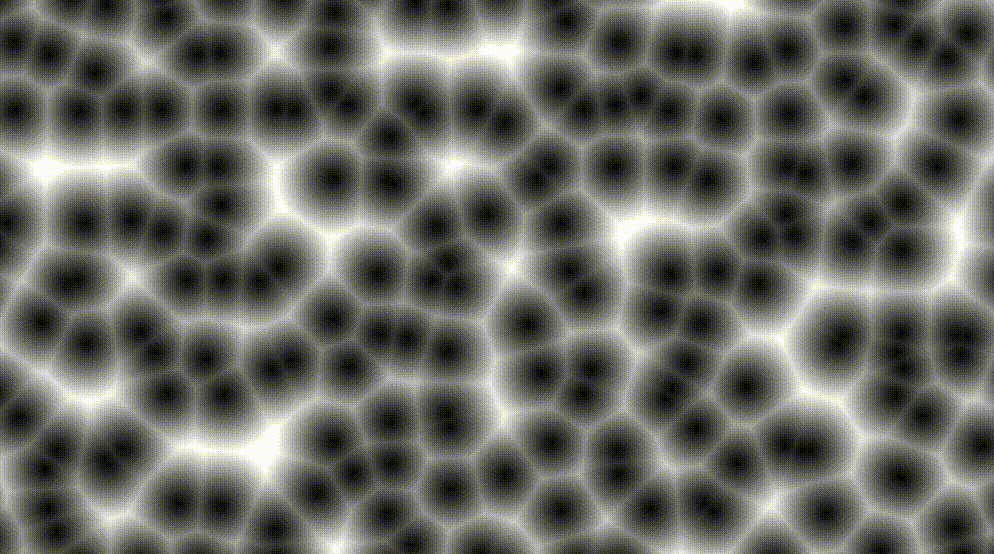uv *= 10.0;

vec2 u = floor(uv);
vec2 f = fract(uv);
float m = 1.0;
vec2 mPos;
for (int y = -1; y <= 1; y++) {
for (int x = -1; x <= 1; x++) {
vec2 p = rand(u + vec2(x, y));
// Just gotta add one single line here
p = 0.5 + 0.5 * sin(1.23 * time + 10.1 * p);
// ^^^^^^^^^^^^^^^^^^^^^^^^^^^^^^^^^^^
float d = distance(f - vec2(x, y), p);
if (d < m) {
m = d;
mPos = p;
}
}
}

vec3 color = vec3(rand(mPos), 1.0);

gl_FragColor = vec4(
color,
1.0);


## Conclusion

Well, we are ending things here. I’ve truly learned some interesting effects today. It could fake 3D balls effect, without actually Ray marching; you know that’s hard. We could also just use it like regular noise, even though Worley noise has less application than Perlin noise. There are usages for it, of course, and I hope one day they could actually come in handle!

]]>
LuaPT Showcase: Week 62023-09-16T22:45:30+08:002023-09-16T22:45:30+08:00https://blog.42yeah.is/rendering/2023/09/16/luapt-6
• Week 1: project introduction and high-level design
• Week 3: rudimentary UI and job dispatch system
• Week 4: true multithreaded Lua)
• Week 5: LuaJIT and BVH
• Week 6: you are here
• Hi! Things have been busy for me. I have been trying to lock onto computer graphics jobs, so if any of my 3 viewers know some job openings, please let me know. Also, I appreciate your presence. Truly I do.

Back to the main topic. I don’t have much time this week; so I spent them on rendering Spot, optimizing BVH construction, and adding a few things here and there (for example, adding uv interpolation, and rejection-sampling ray generataion.) I have also added texture sampling so now the renderings don’t have to be made out of crystals anymore. Let’s take a look at some of them!

## GI Spot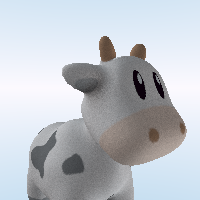First we see our dear global illuminated Spot. In the current state however, since we don’t have a proper material system in place, this is simply rendered via a two-bounce low-budget fake GI method:

1. Rays are generated and traced from the camera.
2. Upon intersecting with a triangle, 5 new rays are reflected from the surface (surfaces are always Lambertian.)
3. Now trace these 5 rays. When they intersect with a triangle, we calculate its brightness via the Phong shading model (without specular). If it doesn’t, we sample the sky brightness over there.
4. We average over the brightness these 5 rays bring back, and combine that with the texture color to produce the final color.

After gamma correction, a matte-looking Spot is produced. I think that’s kind of cute!

## Cornell Box (With Balls!)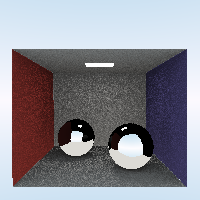Here’s the Cornell Box rendered using the same approximate method. You can see the brightness calculated from the Phong shading model in the reflection of the balls. There is also some kind of light leaking around the edges. To increase realism (because the light source is kind of small here,) the second-bounce sample size is increased to 100 (except the ball reflection - that’s still 1.)

## Sponza!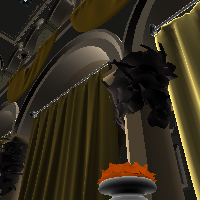This Sponza model is downloaded from here and man, it’s huge. Even fake GI is too much for LuaPT right now; and I think there’s also something wrong with the textures at the moment. I have tried rendering this scene with the same parameters as the cornell box, and it took me 30 full minutes with unsatisfactory result (I have tuned the samples down to 5 - don’t think that 100 samples are doable.)

## That’s It For Now

So, that’s where we are at the moment. No big improvements this week, but a few QoL changes here and there. Maybe later down the line, I will turn this into a proper open source project by having a README.md and force all my friends to star it. My current plan is to implement BxDF in the short, forseeable future; due to the immense time needed to render Sponza, I guess you guys won’t be seeing it for another good while. So that’s all guys. See you next time!

You can check out the source code here.

]]>
Writing a Pathtracer in Lua, Week 5: LuaJIT and BVH2023-09-09T22:10:30+08:002023-09-09T22:10:30+08:00https://blog.42yeah.is/rendering/2023/09/09/luapt-5
• Week 1: project introduction and high-level design
• Week 3: rudimentary UI and job dispatch system
• Week 4: true multithreaded Lua)
• Week 5 (you are here)
• Hi! This week marks a great change for LuaPT. To further accelerate the tracing process, multiple things have been optimized and changed, and some are rebuilt from the ground up. This week, we will be introducing LuaJIT, and most importantly, the ffi extension. After that, we will implement a BVH acceleration structure in full Lua (or at least 80% Lua). LuaJIT has accelerated Lua so much and ffi has greatly simplified C++/Lua interoperation, I don’t even know where to begin. So let’s begin!## LuaJIT

I have started trying improving the speed of LuaPT since week 4. Since week 3 begins LuaPT’s performance has been rapidly degrading and I know that soon enough, it will be so slow that I might as well trace the rays by hand. But this is when LuaJIT comes to the rescue. By simply replacing my exisitng Lua library and linking LuaJIT instead I can already see a significant increase in speed.

Next, by using ffi extension I ported my whole math library to C++.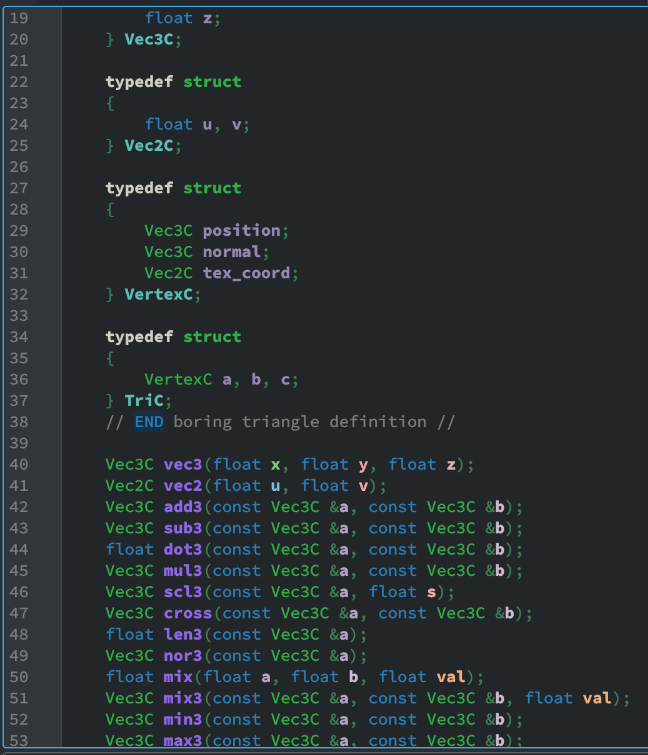As models & meshes are represented using a continuous glm::vec3 vector, we can exploit this fact and force a pointer cast when the Lua script is asking for triangles. Vector math in C++ is magnitudes faster than in Lua, and by doing so I achieved another speed up.

The ffi library is so cool. I just need to implement the functions in C++ (with an extern C block), then copy & paste my header into a ffi.cdef block. Now I can just call these functions in Lua. It’s crazy how convenient it is.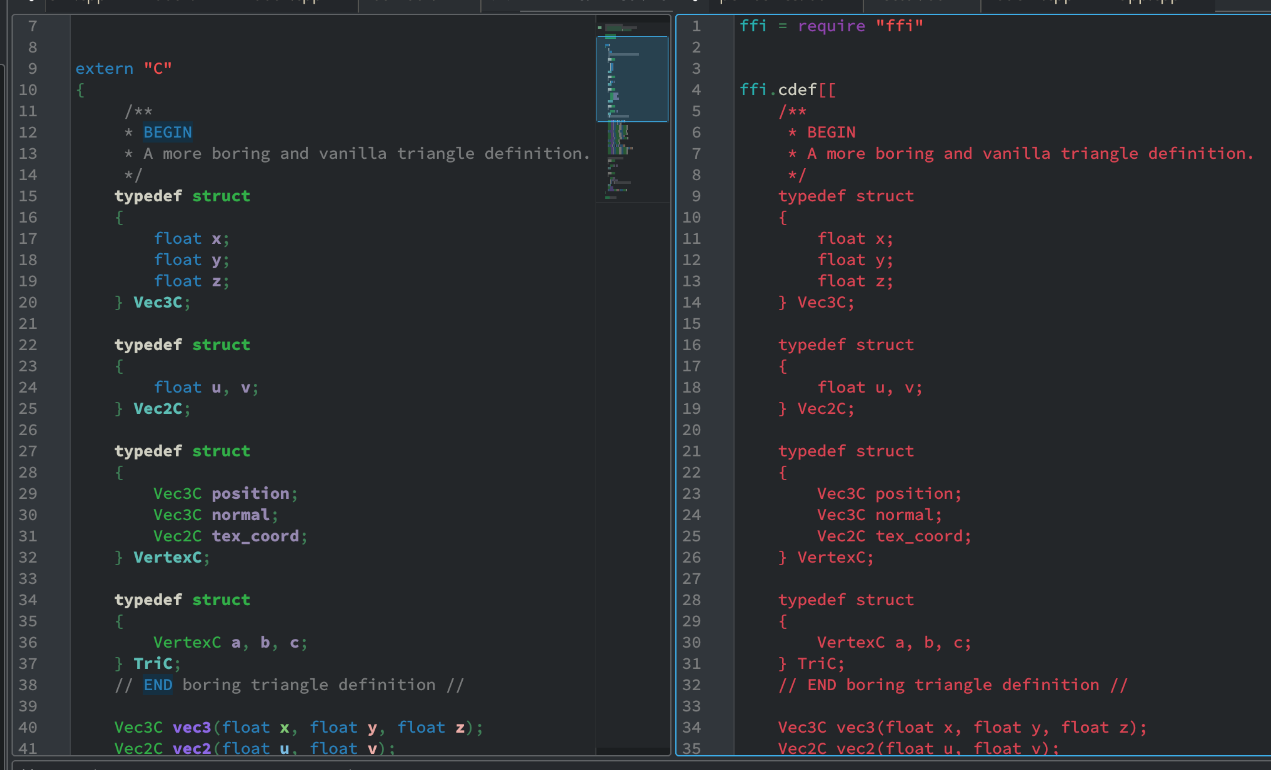The switch to LuaJIT is time-consuming but nothing too technical. All the previous methods and APIs (including Image and Model) ported onto LuaJIT, however losing their object-oriented APIs in the process. But I think it’s a rather small sacrifice to obtain the Speed. If you are interested in learning how LuaJIT works, I strongly recommend you check out the official website. It’s short, concise, and in less than 10 lines of code showed me how I can use it. It’s awesome. So after deprecating the Lua library and switching to LuaJIT, I begin working on a few other improvements.

## Intersection

### Ray-Box Intersection

As we will be implementing a BVH, we have to implement a ray-box intersection method first. Here we will make use of the one in Scratchapixel, aka the slab method.

function intersect_box(ro, rd, box)
local rdinv = vec3(1 / rd.x, 1 / rd.y, 1 / rd.z)
local tmin = -1e309 -- 1e309 is infinity in Lua
local tmax = 1e309

if rd.x ~= 0 then
local tx1 = (box.min.x - ro.x) * rdinv.x
local tx2 = (box.max.x - ro.x) * rdinv.x

tmin = math.max(tmin, math.min(tx1, tx2))
tmax = math.min(tmax, math.max(tx1, tx2))
end
if rd.y ~= 0 then
local ty1 = (box.min.y - ro.y) * rdinv.y
local ty2 = (box.max.y - ro.y) * rdinv.y

tmin = math.max(tmin, math.min(ty1, ty2))
tmax = math.min(tmax, math.max(ty1, ty2))
end
if rd.z ~= 0 then
local tz1 = (box.min.z - ro.z) * rdinv.z
local tz2 = (box.max.z - ro.z) * rdinv.z

tmin = math.max(tmin, math.min(tz1, tz2))
tmax = math.min(tmax, math.max(tz1, tz2))
end

if tmax >= tmin then
return tmin, tmax
else
return nil
end
end


Of course then we will need a bounding box data structure. The bounding box BBox is first defined in plain C then passed onto Lua using LuaJIT.

typedef struct
{
Vec3C min, max;
} BBox;


### Better Ray-Triangle Intersection

The brute force method of ray-triangle intersection test introduced in week 2 is also starting to slow us down. To counter this issue, I have switched the ray-triangle intersection test to a faster one, namely the Moller-Trumbore method. both the Scotty3D website and Scratchapixel are excellent learning sources.

function intersect_mt(ro, rd, tri, tmin, tmax)
local e1 = sub3(tri.b.position, tri.a.position)
local e2 = sub3(tri.c.position, tri.a.position)

local pvec = cross(rd, e2)
local det = dot3(e1, pvec)

-- Are they almost parallel?
if (math.abs(det) < 0.0001) then
return nil
end

local inv = 1.0 / det
local tvec = sub3(ro, tri.a.position)
local u = dot3(pvec, tvec) * inv
if u < 0 or u > 1 then
return nil
end

local qvec = cross(tvec, e1)
local v = dot3(rd, qvec) * inv

if v < 0 or u + v > 1 then
return nil
end

local t = dot3(e2, qvec) * inv
if t < tmin or t > tmax then
return nil
end

return vec3(u, v, t)
end


To further increase render precision, add more zeros to if (math.abs(det) < 0.0001) then. The implementation of Moller-Trumbore method gave us a slight speed increase for each pixel.

## BVH Construction

And finally, now it’s time for us to construct a BVH. We can’t implement the whole BVH in Lua, because multithreading is achieved through multiple Lua instances and there isn’t an effective way to sync Lua variables between Lua states. So instead, the BVH data structure will be defined in C/C++, with a few getters/setters, but no serious functions that could construct a BVH directly.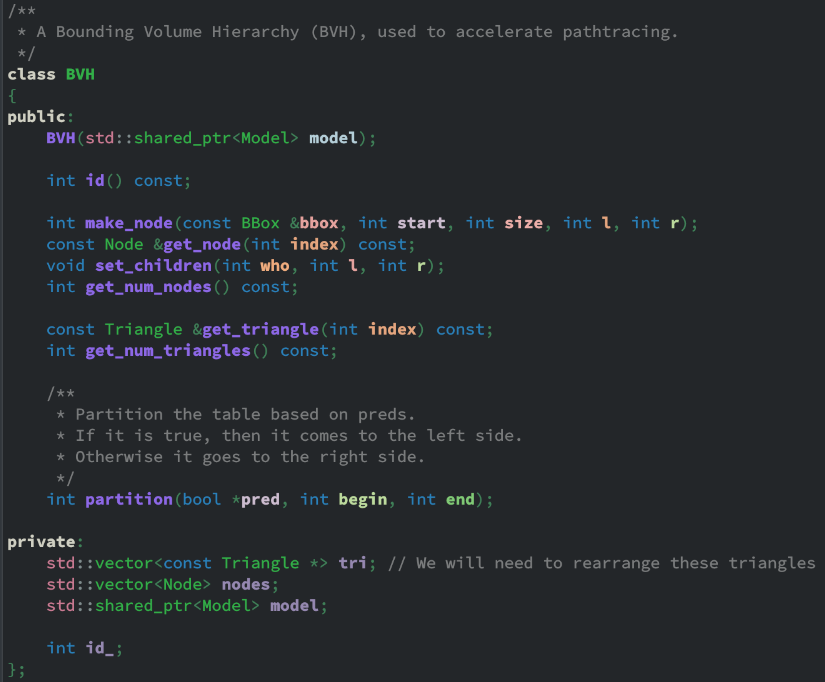### BVH Definition (C++ Part)

The only notable functions are the constructor of BVH and the partition function. We will go over them one by one. In BVH::BVH, we accept a pointer to a Model. The BVH class then copies all triangles into its own array, and creates a root node in the nodes variable. The nodes variable acts like a complete binary tree stored in continuous memory. You can think of it as a heap.

BVH::BVH(std::shared_ptr<Model> model) : model(model)
{
// First make an empty node to fit ALL triangles inside

BBox root_bbox = bbox();
for (int i = 0; i < model->get_num_tris(); i++)
{
TriC *t = model_get_tri(model.get(), i);

// The enclose method encloses a point into a bounding box
enclose(root_bbox, t->a.position);
enclose(root_bbox, t->b.position);
enclose(root_bbox, t->c.position);
tri.push_back(&model->get_triangle(i));
}

make_node(root_bbox, 0, tri.size(), 0, 0);
}


The make_node method is just that - it makes a node, pushes it onto nodes, and return its index. The node is defined as such:

typedef struct
{
BBox bbox;
int start, size;
int l, r; // Left child & right child
} Node;


In this case, we construct a root node for our BVH, make it contain the full triangle array (0 to tri.size()), and its bounding box enclosing all the vertices of the input mesh.

Because BVH stores triangles in whatever order the input mesh give us, we will need some way to rearrange the order of triangles. And to stay true to our project name, Lua has to be the one who does the job. std::partition therefore is out of the question - its final parameter pred requires a lambda function in C/C++. We therefore have to resort to a homemade partition method. By accepting an array of booleans, and putting the true values to the left, false values to the right, we can partition basically anything coming from Lua, with just an extra smidge of memory cost.

int BVH::partition(bool *pred, int begin, int end)
{
assert(begin >= 0 && end < tri.size() && "Invalid partition range");

while (begin <= end)
{
if (!pred[begin])
{
const Triangle *t = tri[begin];
tri[begin] = tri[end];
tri[end] = t;

// Gotta swap that pred as well
bool p = pred[begin];
pred[begin] = pred[end];
pred[end] = p;

end--;
}
else
{
begin++;
}
}
return begin;
}


### BVH Construction (Lua Part)

That’s the whole of C++ part. As you can see, not a lot is going on in there. The real interesting thing happens in Lua.

1. The current (root) node and its bounding box is obtained via the Lua ffi API.
2. For each axis, 8 valid partitions among the bounding box domain is tried.
3. A “Surface Area Heuristic” (SAH) is evaluated for each partition along each domain, and the best one with the lowest score is kept.
4. Finally revert all the changes and execute the partition method with the best SAH.
5. The mesh is now split into 2 following the partition; make 2 new nodes and for each of them, either make leaf or continue partitioning (back to step 1).

Demonstrated below. First, we find the perfect partition with the lowest SAH score: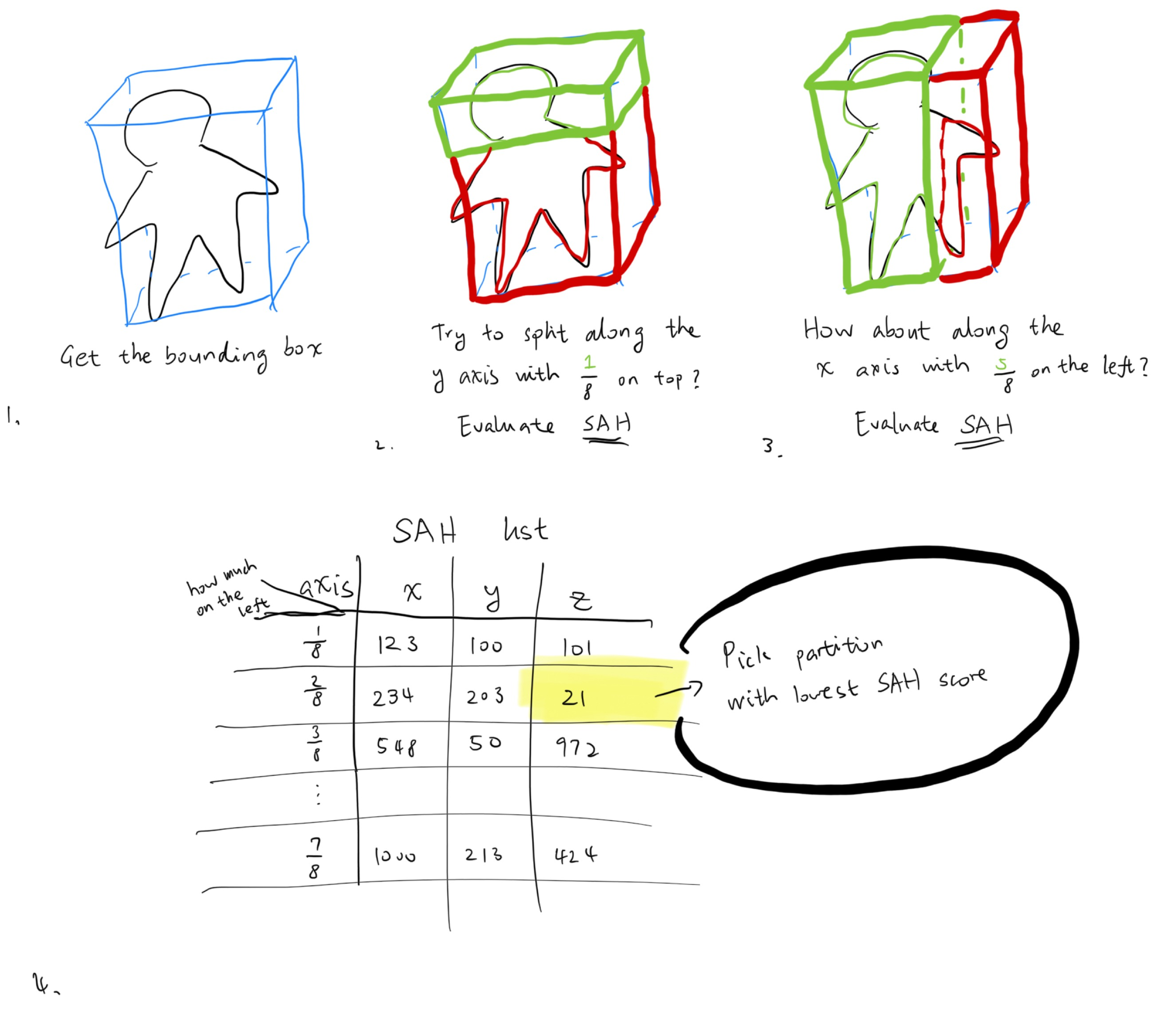Then, we partition the triangles within the node accordingly. Then, we split the array and give them to two new children, and recurse to obtain the best partition for them. This goes on until there aren’t enough triangles to partition, or the best partition is no partition. Then we stop.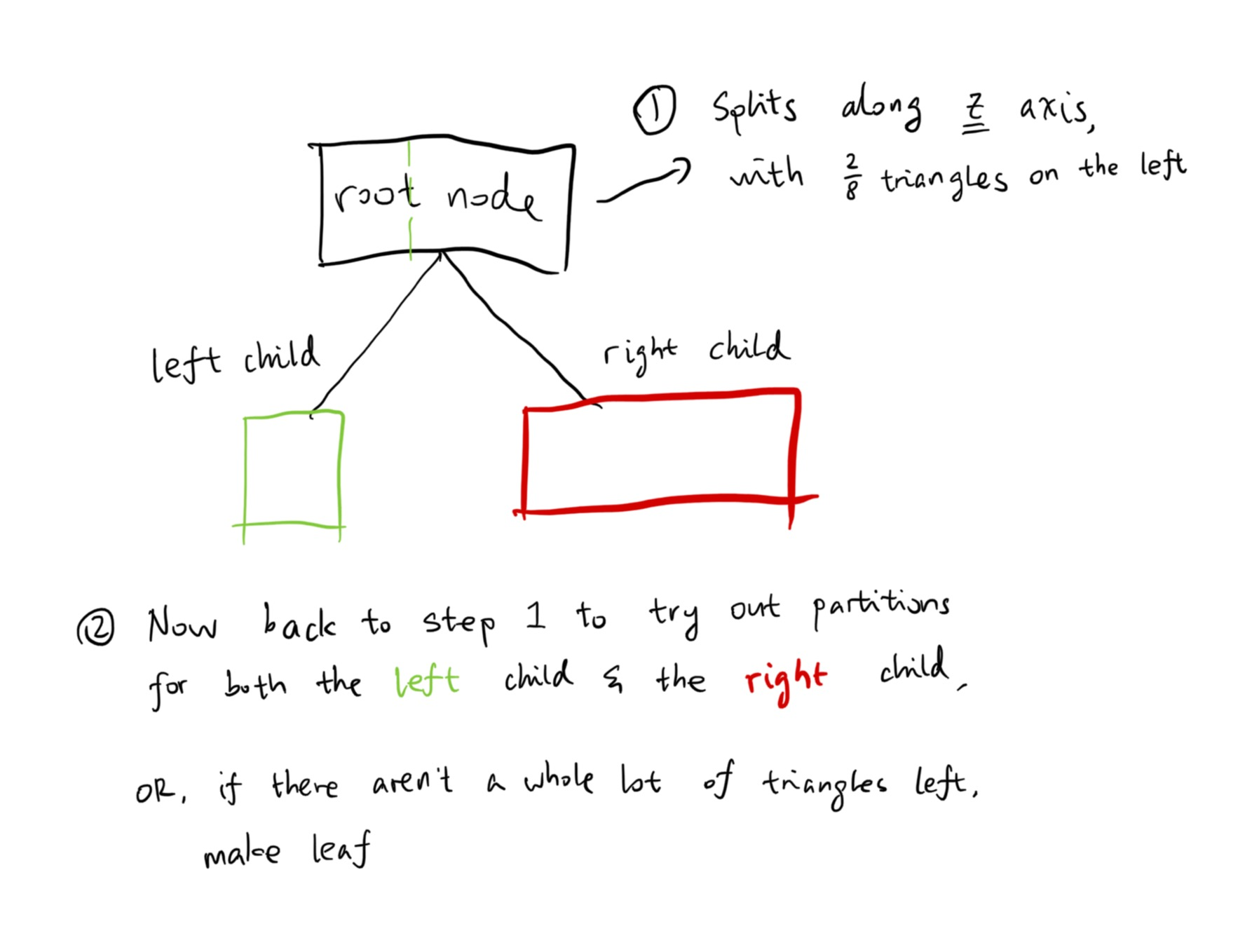For us, the SAH equation is

$\text{SAH} = C_\text{trav} + \frac{S_\text{left}}{S} N_\text{left} C_\text{isect} + \frac{S_\text{right}}{S} N_\text{right} C_\text{isect}$

In which $$C_\text{trav}$$ (the travel cost) is a constant of 1 and the intersect cost $$C_\text{isect}$$ is 2. The $$\frac{S_\text{left}}{S}$$ and $$\frac{S_\text{right}}{S}$$ are simply the split ratio (the $$\frac{k}{8}$$ above).

This is how that looks in Lua:

-- First call the BVH constructor with a model
local bvh = make_bvh(model)

function determine_side(p, offset, axis)
if axis == 0 then
return p.x < offset.x
elseif axis == 1 then
return p.y < offset.y
else
return p.z < offset.z
end
end

function determine_area_ratio(poff, span, axis)
if axis == 0 then
return poff.x / span.x, (span.x - poff.x) / span.x
elseif axis == 1 then
return poff.y / span.y, (span.y - poff.y) / span.y
else
return poff.z / span.z, (span.z - poff.z) / span.z
end
end

function bvh_construct(node_idx, start, fin)
if fin - start + 1 <= 8 then
-- No need to make BVH; not a lot of triangles here
return
end

local n = bvh_get_node(bvh, node_idx)
local span = sub3(n.bbox.max, n.bbox.min)

-- split them into 8 buckets
local num_buckets = 8
local split_step = scl3(span, 1 / num_buckets)

-- Bests
local best_axis = 0
local best_step = 1
local best_sah = 1e309
local best_offset = 0

for axis = 0, 2 do
-- Tentatively partition them along these axis

for step = 1, 7 do
-- 1. Somehow partition it.
local table = make_partitioning_table(bvh)
local poff = scl3(split_step, step) -- Plane offset
for i = start, fin do
local tri = bvh_get_tri(bvh, i)

table[i] = determine_side(centroid, plane, axis)
end
local offset = partition(bvh, table, start, fin)

-- 2. Calculate SAH. Record the best one.
local left, right = determine_area_ratio(poff, span, axis)
local sah = 1 + 2 * left * (offset - start) + 2 * right * (fin - offset + 1)
if sah < best_sah then
best_sah = sah
best_step = step
best_axis = axis
best_offset = offset
end
end
end

-- That's not very constructive.
if best_offset == start or best_offset == fin + 1 then
return
end

-- Partition using the best one.
local table = make_partitioning_table(bvh)
local poff = scl3(split_step, best_step)
for i = start, fin do
local tri = bvh_get_tri(bvh, i)

table[i] = determine_side(centroid, plane, best_axis)
end
local offset = partition(bvh, table, start, fin)

local left_box = bbox()
local right_box = bbox()
for i = start, offset - 1 do
local tri = bvh_get_tri(bvh, i)
enclose(left_box, tri.a.position)
enclose(left_box, tri.b.position)
enclose(left_box, tri.c.position)
end
for i = offset, fin do
local tri = bvh_get_tri(bvh, i)
enclose(right_box, tri.a.position)
enclose(right_box, tri.b.position)
enclose(right_box, tri.c.position)
end

local l = bvh_push_node(bvh, left_box, start, offset - start, 0, 0)
local r = bvh_push_node(bvh, right_box, offset, fin - offset + 1, 0, 0)
bvh_node_set_children(bvh, node_idx, l, r)

-- Recurse into l and r ???
bvh_construct(l, start, offset - 1)
bvh_construct(r, offset, fin)
end

-- Construct BVH for the whole thing
bvh_construct(0, 0, bvh_tri_count(bvh) - 1)


## Current Result

With the shiny new BVH in place, combined with LuaJIT, our render is now much, much faster. Compared to a non-BVH (yes-LuaJIT) rendering of Spot the cow, which can take up to 10 minute, BVH-enabled version of the same scene now only takes 2 and a half minutes.Here’s the Blender monkey Suzanne:Aaaand here’s a 720p torus.If you have you missed it, here’s the link to the source code. Hit a star if you feel like it. It will always shoot a stream of dopamine straight to my brain. But in any case, I will see you next week!

]]>
Writing a Pathtracer in Lua, Week 4: True Multithreaded Lua2023-09-02T17:18:30+08:002023-09-02T17:18:30+08:00https://blog.42yeah.is/rendering/2023/09/02/luapt-4Previous weeks:

Hello September! This is week 4 of me trying to write a pathtracer in Lua (with no pathtracer… yet.) This week, we will build upon what we have achieved last week to make a fully functional multithreading model, so that things can actually speed up.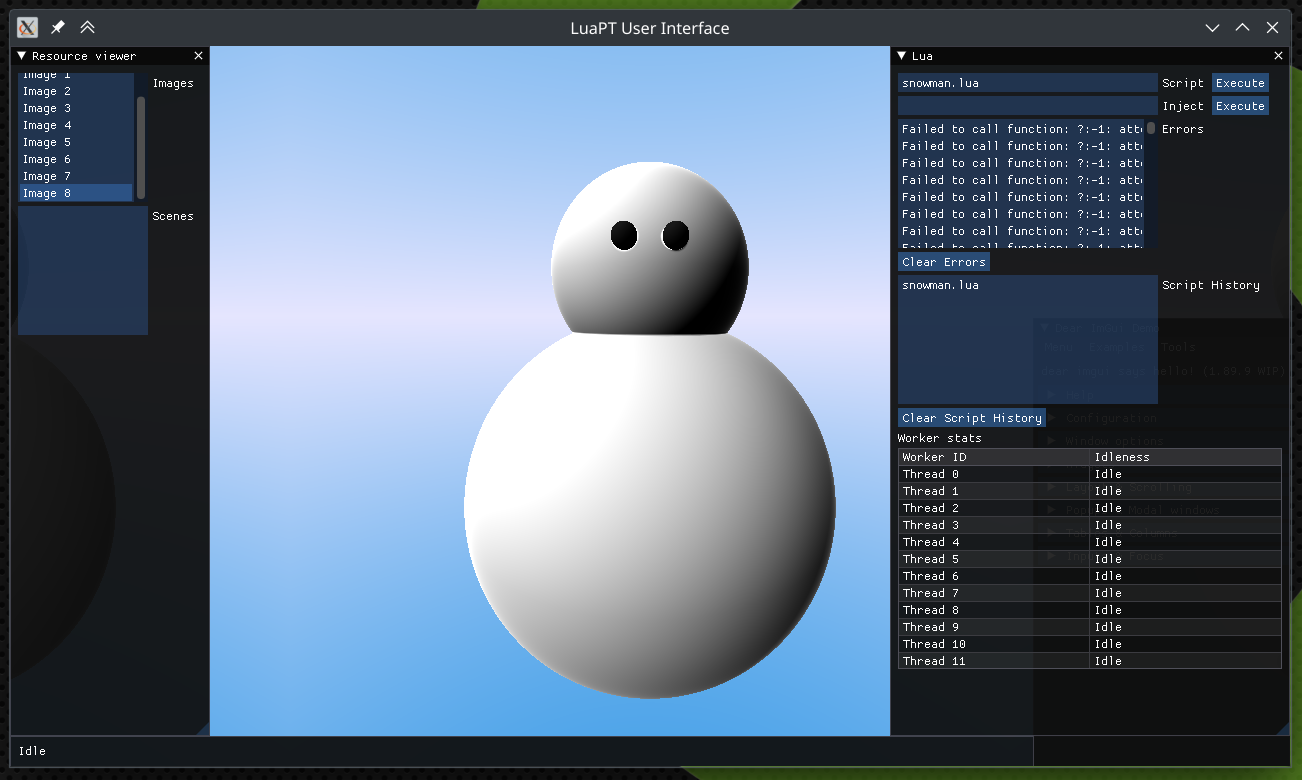## Job Queue

Although Lua has something called “coroutine”, they are merely lightweight threads, and does not actually run simultaenously. Therefore, coroutine does not exploit the benefit of having multiple CPUs, and is not suitable for our needs. What we need is real multithreading support.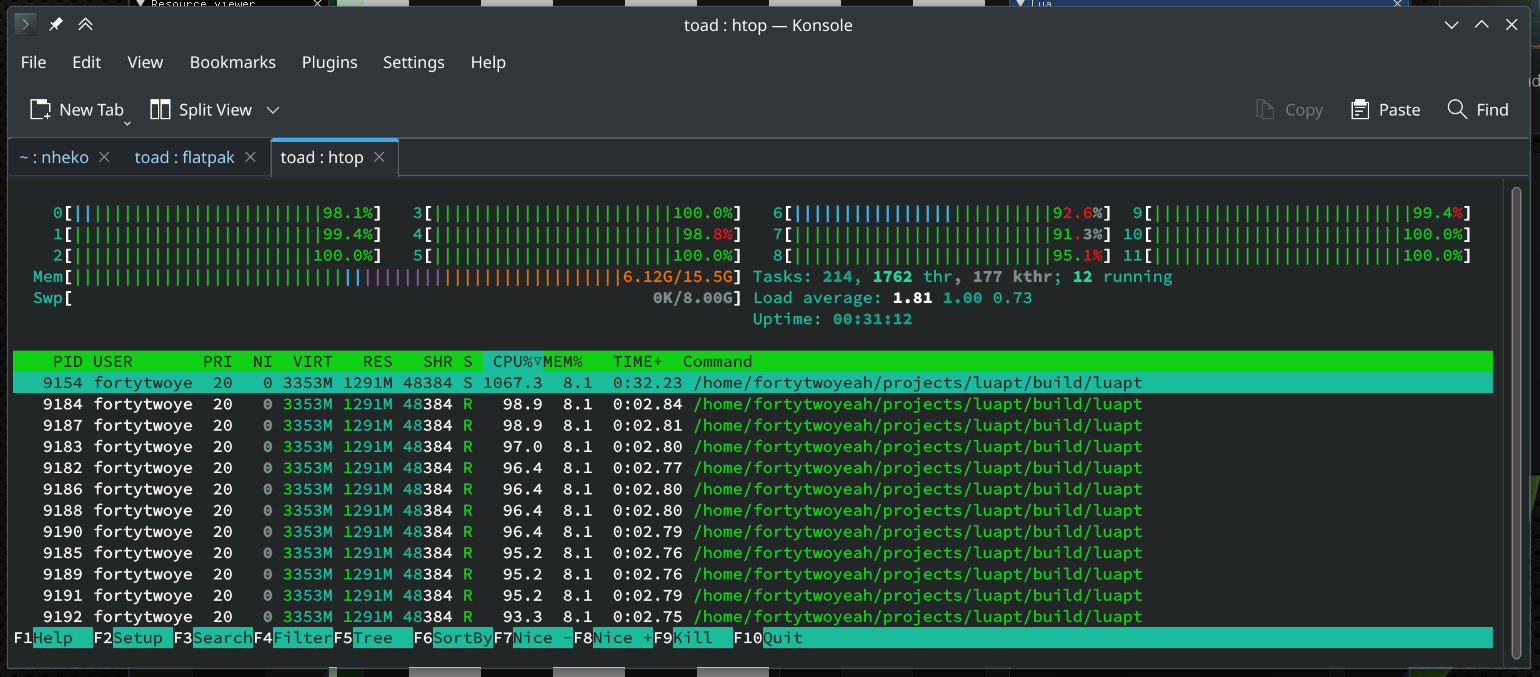There are some real multithreading libraries out there for Lua as well - effil being the most prominent one. However, writing one ourselves will always give us finer-grained control over it. So let’s write one!

Here’s the high-level design. When the application starts up, maximum amount of threads are started with it as well. Then, using a std::condition_variable, these worker threads start waiting for new jobs entering the job queue. Once a job enters the job queue, they start to work, and after that, they revert to being idle again.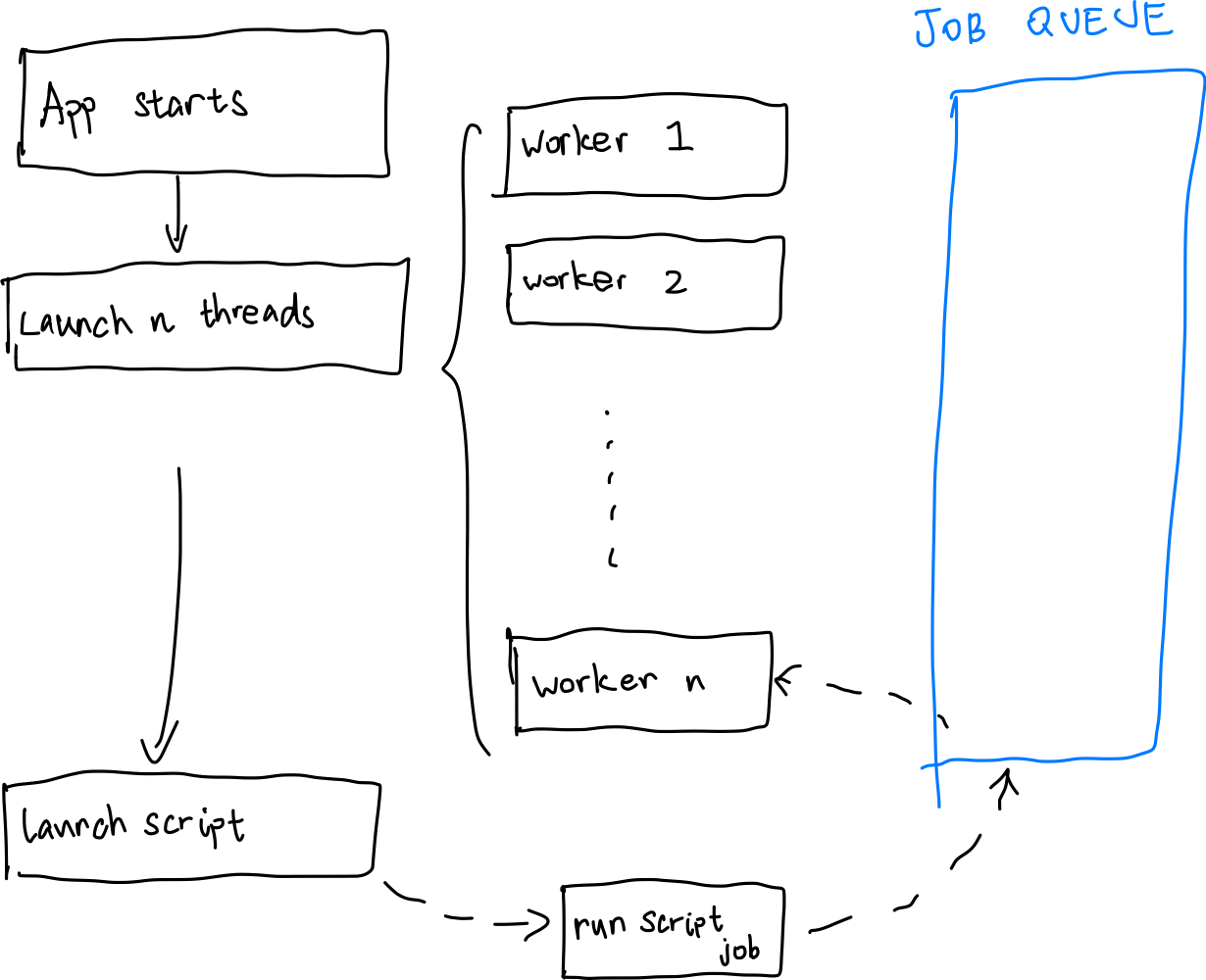You can check out week 3 for an approximate implementation on the worker thread. Workers will execute corresponding work routine according to the job type passed along with the job description. They can either be do nothing, kill thyself, run a script, or execute parallel bytecode. We are going to take a gander at the last one.

## Executing Bytecode

Inside our C++ implementation, we defined a function called shade, requiring the following parameters:

shade(int w, int h, int image_handle, function shade_function)


The image handle points to a Image implemented in C++. You can see its implementation in week 1. We register this function to our Lua state using the Lua C API. When the Lua script calls this function, $$w \times h$$ parallel jobs are pushed into the job queue, with the following job description:

struct ParallelParams
{
float u, v;
int x, y, w, h;
std::string bytecode;
int image_handle;
};


Since the Lua state machine is very fragile when it comes to multithreading, and though lua_newthread does return a new lua_State *, that doesn’t support real multhreading environment in reality (trust me, I tried.) As a result we’ll have to create separate lua_State *s for each worker thread, isolating basically everything. This is also the solution of the effil library.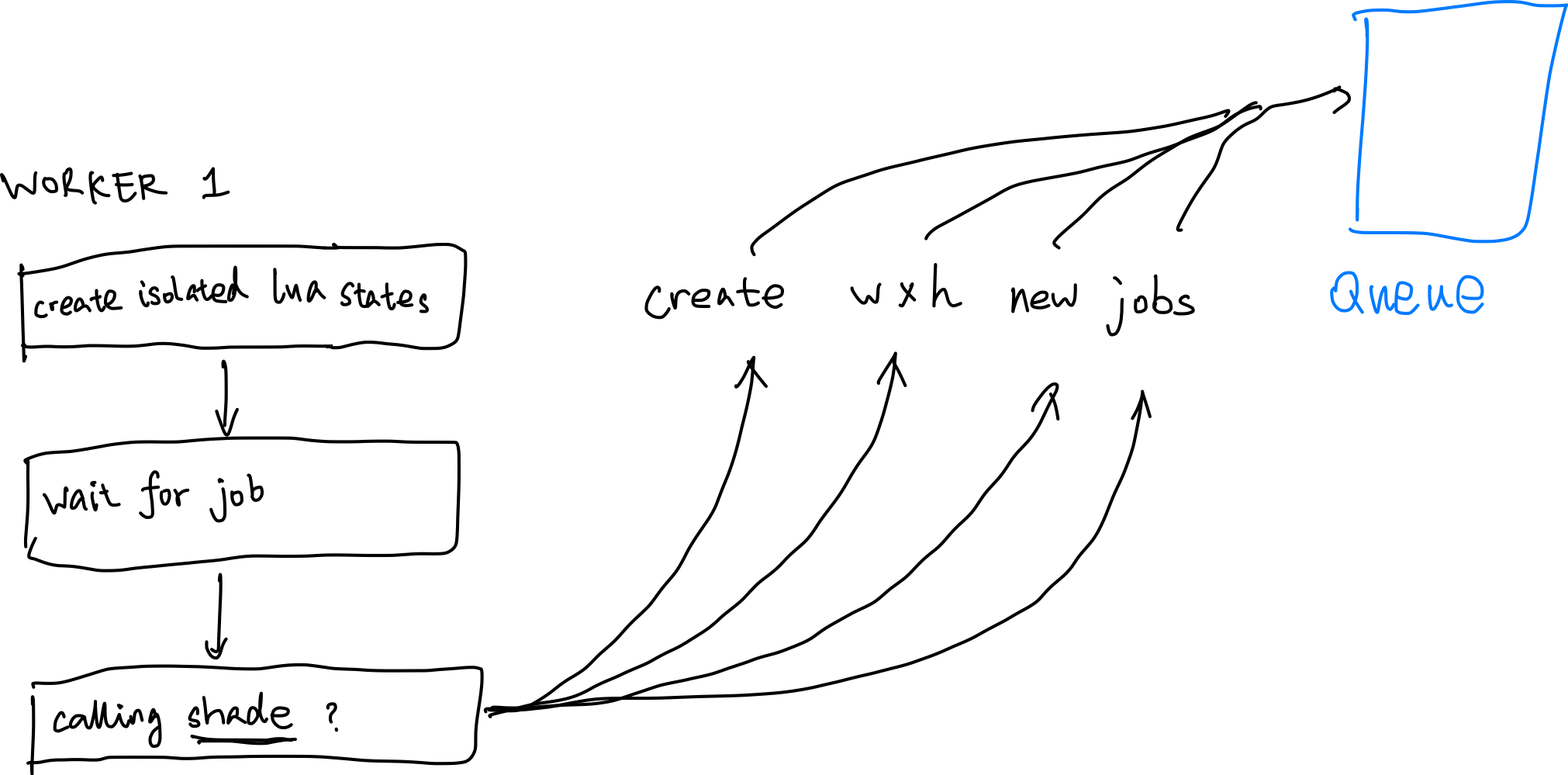But when all Lua states are isolated, the problem now becomes - how do we pass the function defined in one Lua state to another Lua state? To that end, we make use of lua_dump to dump the whole function to a string (in the form of bytecode,) then each worker loads it back up and executes it.

void Lua::call_shade(const std::string &bytecode, float u, float v, int x, int y, int w, int h, int image_handle)
{
if (error)
{
std::stringstream err;
err << "Failed to load bytecode: " << lua_tostring(l, -1);
err_log.push_back(err.str());
}

lua_pushnumber(l, u);
// push all 7 parameters...

if (lua_pcall(l, 7, 0, 0))
{
// pcall failed
}
}


## Current Result

After adding some additional bells & whistles, we can now finally raymarch a 720x720 snowman in acceptable speed. It’s still slow. :(

(And yes, I forgot to close ImGui::ShowDemoWindow.)

Even though concurrency has been achieved, data transportation still poses a great challenge. Right now we hack this by setting the image handle directly as one of the many parameters of the shade user function; but in the future when there are meshes, scenes, and BVHs, we might not be as lucky. My one idea is to use shared tables for all and access can only be done via registered C functions (so not entirely in Lua.) Although it’s not as graceful, I suppose this will further accelerate the render speed so I might go on that path. As always, stay tuned for more!

]]>
Writing a Pathtracer in Lua, Week 3: Rudimentary UI and Parallel Computing2023-08-26T17:02:30+08:002023-08-26T17:02:30+08:00https://blog.42yeah.is/rendering/2023/08/26/luapt-3Welcome to week 3 of trying to write a pathtracer in Lua! If you’ve missed out on week 1 and week 2, definitely check them out first! This week there won’t be any Lua; we will get some rudimentary UI and parallel computing into our little pathtracer, so that hot reloading becomes much more convenient and cool. And although the project is far from finished and usable, it is open sourced now so you guys can take a look!

## User Interface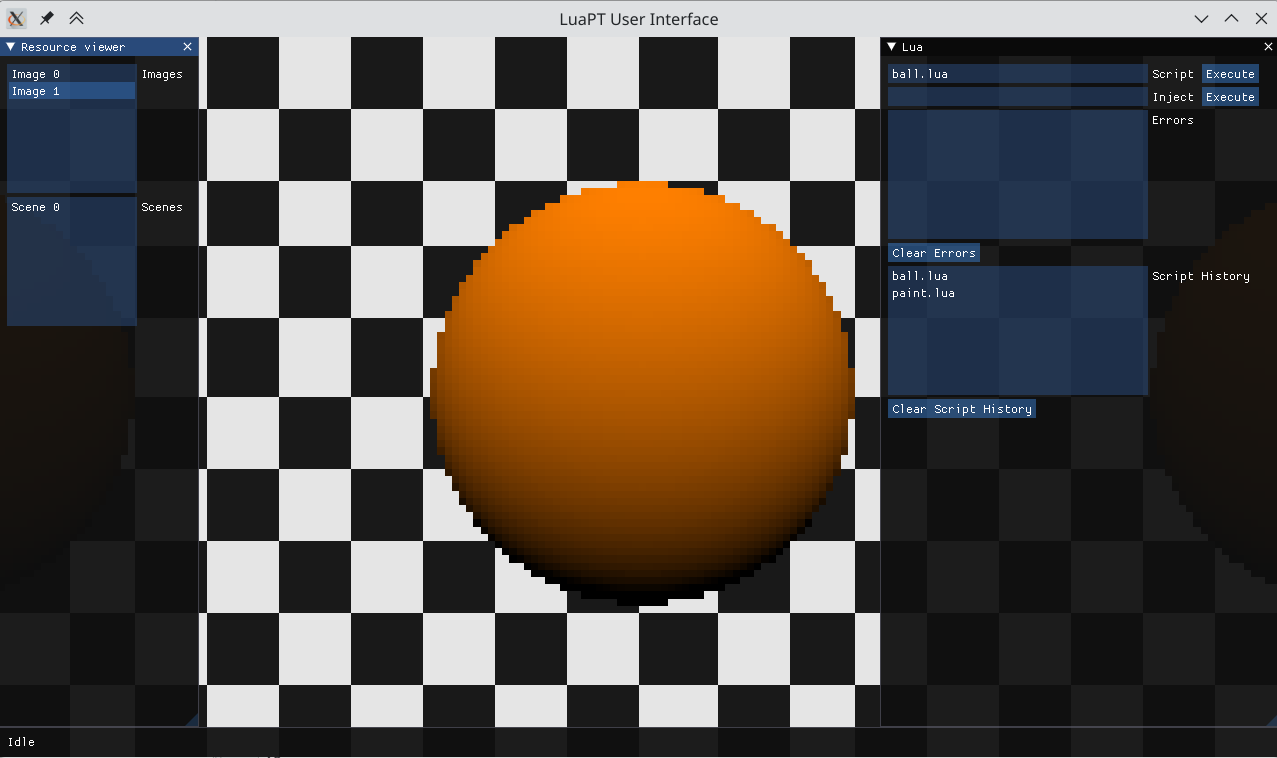The user interface is, of course, implemented using the awesome ImGui library. The left sidebar can view the images & models Lua created & loaded; and Lua scripts and code can be executed on the right sidebar. The bottom bar indicates the current status of the system - whether it’s running a Lua script or not. It’s very crude right now but I believe the user interface will be fleshed out bit by bit in the future.

When proper multi-threading supported is added for Lua, the bottom bar should show a progress bar to indicate the current progress - at least that’s what I wish. I won’t be going into much detail on designing the UI - if you want to learn about ImGui, the best way is to download the library and read the code in ImGui::ShowDemoWindow() by yourself.

With the introduction of UI, we now have to get basic multithreading support done as well - we don’t want a complex Lua script to lag the whole app. Instead, when a Lua script is running, we want to know about the current status, and what’s being changed. That would be so cool. So first, the application will launch a bunch of worker threads after all other resources are being properly loaded:

int con = std::thread::hardware_concurrency();
if (con == 0)
{
std::cerr << "WARNING: No hardware hardware concurrency? One thread will be launched regardless to separate Lua from UI." << std::endl;
con = 1;
}
for (int i = 0; i < con; i++)
{
}


We try to launch as many thread as possible to consume maximum CPU. worker_thread is a static method which constantly waits for new available jobs. And when it comes, it takes the job, executes it, and then wait again.

void App::worker_thread(App &app, int thread_id)
{
while (app.alive)
{
// app.mu is a std::mutex.
std::unique_lock<std::mutex> lk(app.mu);

// app.cv is a condition variable.
app.cv.wait(lk, [&]()
{
// if there are available job, or the app is quitting, then aquire lock.
return !app.jobs.empty() || !app.alive;
});

if (!app.alive)
{
// Time to kill thyself
return;
}

// Take a job. Any job.
Job job = app.jobs.front();
app.jobs.pop();
lk.unlock();

switch (job.get_job_type())
{
case JobType::Nothing:
break;

case JobType::Suicide:
return;

case JobType::RunScript:
// Lua::inst() is the singleton instance of the Lua state machine.
Lua::inst()->execute_file(job.get_script_path());
break;

case JobType::Execute:
Lua::inst()->execute(job.get_code_injection());
break;
}

{
std::lock_guard<std::mutex> lk(app.mu);
app.done_job_count++;
}
}
}


A worker waits for a job by first aquiring a lock, then releases that lock to put it under the control of a condition variable. The lock will be aquired again when the job queue’s not empty (!app.jobs.empty()) or the app is quitting to properly terminate itself. Four types of jobs are supported.

1. Doing absolutely nothing.
2. Terminate itself.
3. Run a script.
4. Execute a piece of code.

The Job class is very trivial and is defined as such:

class Job
{
public:
// Constructors, destructors, getters, setters, etc...

private:
JobType type;
std::string script_path;
std::string code_injection;
};


When the user tries to run some sort of script by pressing on the Execute button, a new job is queued and a single worker is notified:

void App::queue_single_job(const Job &job)
{
{
std::lock_guard<std::mutex> lk(mu);
batch_job_count = 1;
done_job_count = 0;
jobs.push(job);
}
cv.notify_one();
}


If, in the future, true parallel computing is supported, then our batch_job_count can be wayy larger than 1. The user interface will update accordingly to check if Lua is busy right now.

## Image Viewing

Recall what we have done on week 1; when Lua creates an Image, it is conveniently stored in a vector accessible by our C++ code. This makes it very convenient to view the images at runtime. And to do so, we need a little bit of OpenGL. We need to first create a wrapper class ImageGL:

class ImageGL
{
public:
// Constructors & desctructors
// ...

bool import_from_image(const Image &image);
const std::shared_ptr<Image> get_base_image() const;
void bind() const;

private:
bool initialized;
GLuint texture;
std::shared_ptr<Image> image;
};


During runtime, the user can select on whatever image their Lua script has created. OpenGL then renders that image by first uploading the image to GPU, and then drawing a textured rectangle.

bool ImageGL::import_from_image(const Image &image)
{
initialized = false;
if (texture == GL_NONE)
{
glGenTextures(1, &texture);
glBindTexture(GL_TEXTURE_2D, texture);
glTexParameteri(GL_TEXTURE_2D, GL_TEXTURE_MAG_FILTER, GL_NEAREST);
glTexParameteri(GL_TEXTURE_2D, GL_TEXTURE_MIN_FILTER, GL_NEAREST);
glTexParameteri(GL_TEXTURE_2D, GL_TEXTURE_WRAP_S, GL_REPEAT);
glTexParameteri(GL_TEXTURE_2D, GL_TEXTURE_WRAP_T, GL_REPEAT);
}

glTexImage2D(GL_TEXTURE_2D, 0, GL_RGBA, image.w, image.h, 0, GL_RGBA, GL_UNSIGNED_BYTE, image.get());
initialized = true;

return true;
}


The import_from_image is called per-frame to update the image to GPU. Fortunately, we won’t be needing to repeatedly create the texture again, and glTexImage2D is fairly quick. I guess.

This also has one extremely useful property. That is, we can see the final image being generated in real-time.

## Conclusion

That’s it for this week. Sorry for the lack of progress - I’ve been kind of lazy this week. But up next, we will definitely implement more and more functionality for our LuaPT.

One big problem I’ve encountered is the Lua state, and its inability to multithread. Multithreading wrecks it up real good and usually ends in a panic. By searching on the Internet I’ve found effil, a multithreading library for Lua - but since we have multithreading implemented ourselves we will probably not use it. We will however use its core idea, achieving multithreading by creating multiple Lua states at once and sync their data - so we have that going for us. As always, stay tuned for more!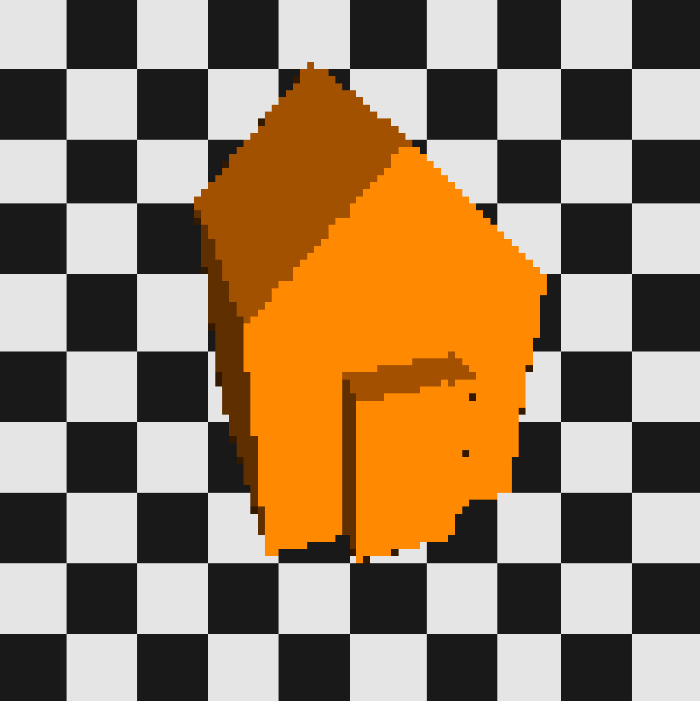]]>
Writing a Pathtracer in Lua, Week 2: Model Loading &amp; Intersection2023-08-19T22:20:30+08:002023-08-19T22:20:30+08:00https://blog.42yeah.is/rendering/2023/08/19/luapt-2Times flies and now we are in week 2 trying to write a pathtracer in Lua. Please check out Week 1 for high-level project overview, thoughts on C++/Lua interoperation, and more. This week, we will first implement model loading in C++, wrap it up into Lua, then use that model loading function to trace some cubes. Let’s go!

We will first make a simple scene class Model to represent the scene:

struct Vertex {
glm::vec3 position;
glm::vec3 normal;
glm::vec2 tex_coord;
};

struct Triangle {
Vertex a, b, c;
};

/**
* The scene representation (for now.)
*/
class Model
{
public:
/**
* Default constructor
*/
Model();

/**
* No copy constructor.
*/
Model(const Model& other) = delete;

/**
* Destructor
*/
~Model();

int id() const;

bool load(const std::string &path, const std::string &mtl_base_dir = "");

int get_num_verts() const;
int get_num_tris() const;

const Triangle &get_triangle(int index) const;

private:
int id_;
bool initialized;
std::vector<Triangle> tri;
};


The scene is made of triangles and for simplicity’s (laziness) sake, we will only support triangular meshes. The load method loads in the scene using tinyobjloader. It’s such a cool & fast library. If you want to learn more about it, I have written a blog post on using tinyobjloader as well.

As we can see right now not a lot is going on. There’s no support for light emitters, BRDFs, etc. Just triangles stored in memory. We will expand this class in the future but as of right now, those are what we have.

After implementing the methods in Model, we wrap them up so that they become Lua functions. For example:

int Lua::make_model(lua_State *l)
{
const char *path = lua_tostring(l, 1);

std::shared_ptr<Model> model = std::make_shared<Model>();
{
return 0;
}

if (!warnings.empty())
{
std::cerr << "WARNING! " << warnings << std::endl;
}

inst()->models.push_back(model);
lua_pushinteger(l, model->id());
return 1;
}


The craziest one to wrap so far is the Triangle::get_triangle function, as it requires returning 24 whole numbers:

int Lua::model_get_tri(lua_State *l)
{
int id = lua_tointeger(l, 1);
int index = lua_tointeger(l, 2);

auto it = // locate triangle using id

const Triangle &tri = (*it)->get_triangle(index);
if (index < 0 || index >= (*it)->get_num_tris())
{
luaL_error(l, "Triangle index out of bounds: %d.", index);
return 0;
}

lua_pushnumber(l, tri.a.position.x);
lua_pushnumber(l, tri.a.position.y);
lua_pushnumber(l, tri.a.position.z);
lua_pushnumber(l, tri.a.normal.x);
// ... this goes on for a while


And now we move onto the Lua side. The premise is basically the same to the Image we mentioned in the previous post. After defining a __gc function for Lua, Lua will now automagically frees the model after it becomes stale.

Model = {
handle = 0,
path = "",
__gc = function()
free_model(self.handle)
end
}

function Model:new(path)
local ret = {}
setmetatable(ret, self)
self.__index = self
ret.path = path
ret.handle = make_model(path)
return ret
end

function Model:tri_count()
return model_tri_count(self.handle)
end

function Model:tri(index)
local apx, apy, apz, anx, any, anz, au, av, bpx, bpy, bpz, bnx, bny, bnz, bu, bv, cpx, cpy, cpz, cnx, cny, cnz, cu, cv = model_get_tri(self.handle, index - 1)

local tri = Triangle:new()
tri.a:set(Vec4:new(apx, apy, apz, 1.0), Vec4:new(anx, any, anz, 1.0), Vec4:new(au, av))
tri.b:set(Vec4:new(bpx, bpy, bpz, 1.0), Vec4:new(bnx, bny, bnz, 1.0), Vec4:new(bu, bv))
tri.c:set(Vec4:new(cpx, cpy, cpz, 1.0), Vec4:new(cnx, cny, cnz, 1.0), Vec4:new(cu, cv))

return tri
end


The tri function constructs a triangle defined in Lua by using the 24 values the model_get_tri function returned above. I am not too sure about this as the performance might be abysmal (because this will be called MANY times for MANY pixels) but I can think of no better alternatives so far.

With the above wrapping done, we can now load & manipulate the scene entirely in Lua.

## Intersection

Since the whole scene is now accessible in Lua, we can implement the most important thing for any raytracers/pathtracers - the intersection function. The main intersection function I am going for here is the ray-triangle intersection function, as we will only support triangular meshes.

There are many ways to do a ray-triangle intersection, and a lot of excellent online resources are available. If you are interested, I would recommend the article in the Graphics Codex, CMU 15462, and the PBRT book. Here I will use a crude method, which is not particularly quick or efficient, but is kind of intuitive, and gets the job done.

### Ray-plane Intersection

Before knowing whether there is a ray-triangle intersection, we have to know first if the ray intersects with the bounding plane of the triangle. We do this by performing a ray-plane intersection. A plane is defined by

$N^T x = c$

where $$N$$ is the normal direction of the plane. For a flat-shaded triangle, that means just about the normal direction of any of its three vertices. Our ray is define by

$r(t) = o + t d$

So assuming the ray does intersects with the plane, there exists a $$t_0$$ which satisfies the $$N^T r(t_0) = c$$.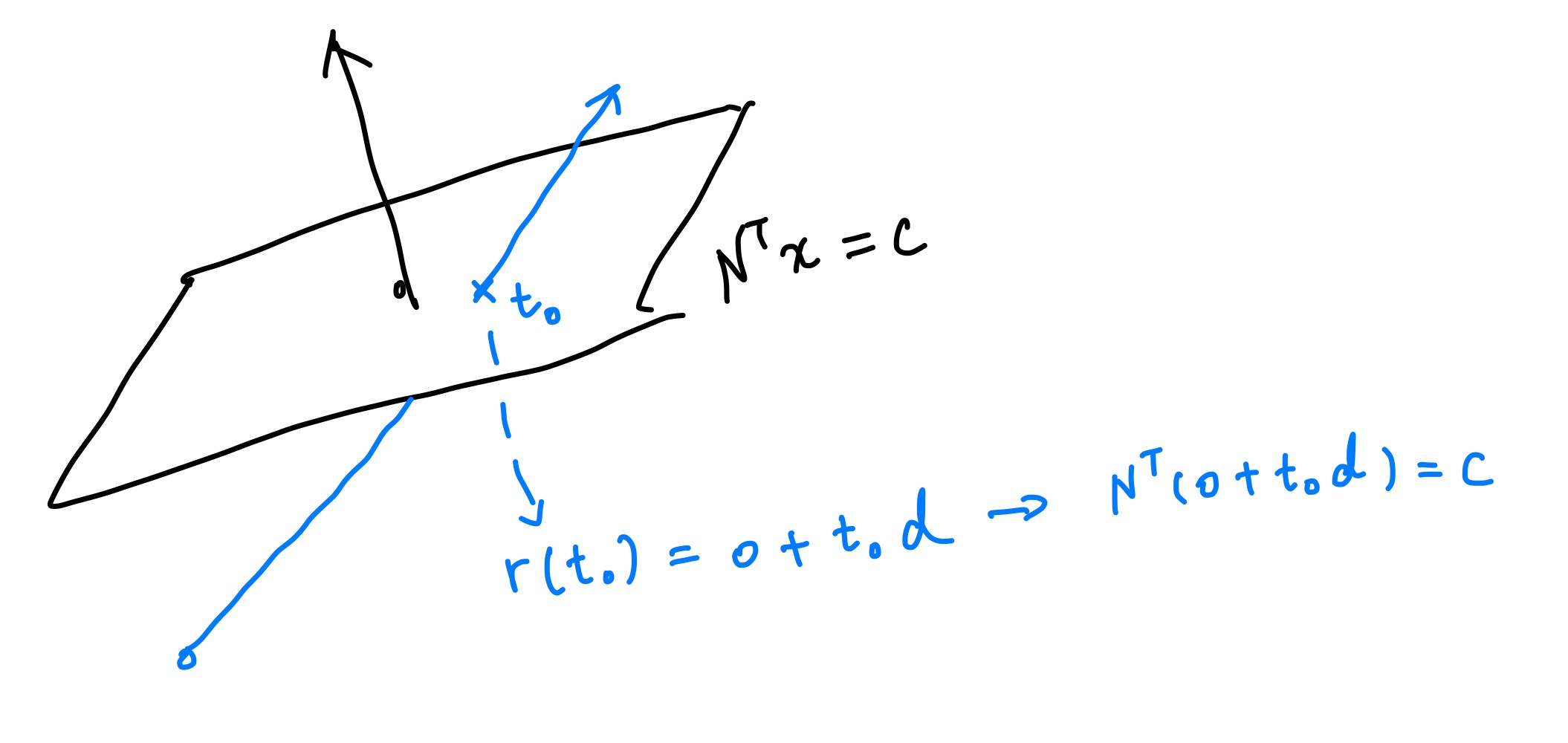If we expand it and solve for $$t_0$$ we get

$t = \frac{c - N^T o}{N^T d}$

This also means that when ray direction d is parallel to normal N, the intersection will be at the infinite point - and therefore there won’t be an intersection.

Our intersect function is defined as such. It accepts a ray origin, a ray direction, and a triangle. We also need to specify tmin and tmax so the intersection result won’t be way too far from us and won’t be behind the ray origin.

function intersect(ro, rd, tri, tmin, tmax)


First, we plug the position and the normal of a random point on the triangle in, and solve for c:

local c = tri.a.position:dot3(tri.a.normal)


After that, we just Lua-ify the above equation.

-- <N^T, d>
-- if the value is less than some threshold, we assume rd is parallel to N and therefore won't have an intersection
local ntd = tri.a.normal:dot3(rd)
if math.abs(ntd) < 0.001 then
return nil
end

local t = (c - tri.a.normal:dot3(ro)) / ntd
if t < tmin or t > tmax then
return nil
end


When a suitable t is found, that means now we also have the intersected point on the plane ($$p_0 = o + t_0 d$$).

### Ray-triangle Intersection

Now onto determining whether the point is inside the triangle. We do this by calculating the barycentric coordinate of point $$p_0$$. In simple terms, barycentric coordinates are related to the areas of the small triangles that are formed when point $$p_0$$ is inside a triangle. To determine if there’s an intersection, we first calculate the ratios of these small triangles’ areas to the entire triangle’s area. Then we confirm that:

1. The sum of these ratios, which correspond to the barycentric coordinates, is equal to 1;
2. None of these ratios are greather than 1.

If any of them fails, then there is no intersection.We calculate the triangle area by using the length of the cross product of any of the two vectors formed by any verties in a triangle, for the length of the cross product is the area of the corressponding parallelogram: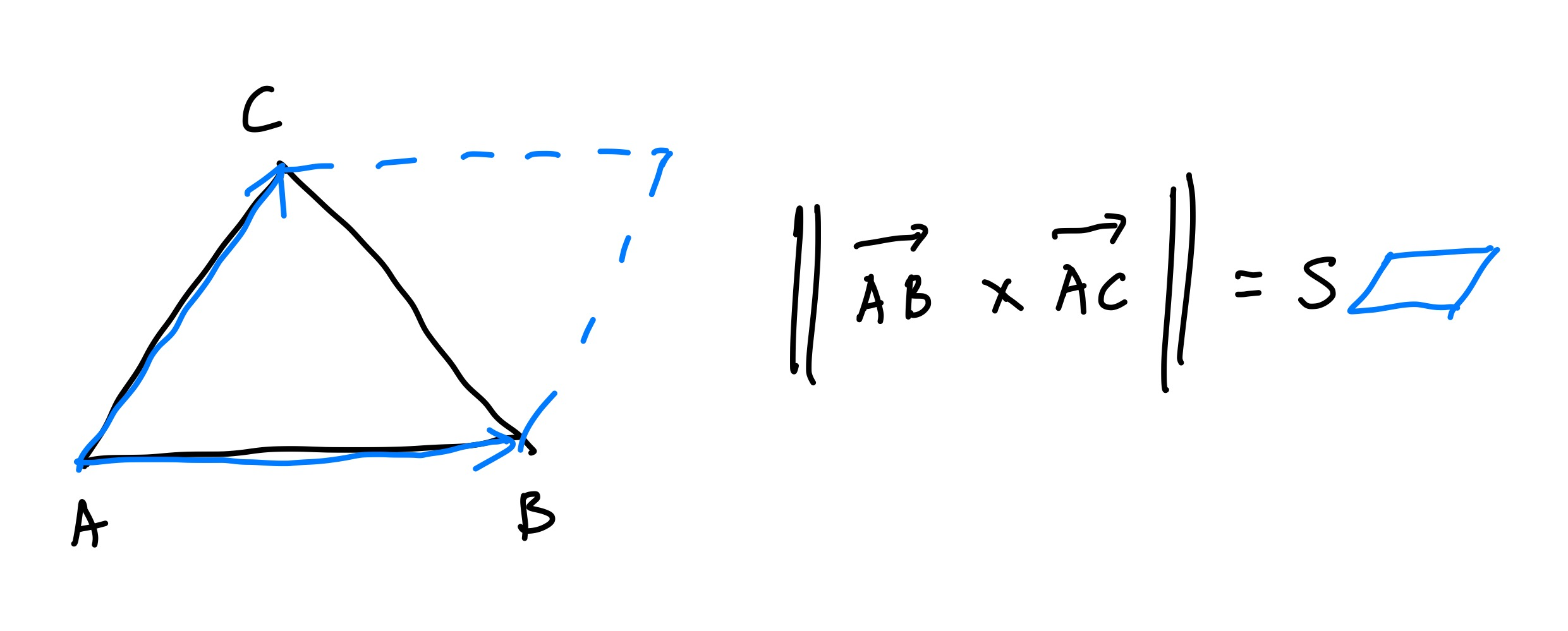Here’s the whole shebang in Lua:

-- ... continued from above

-- If there's an intersection, try to figure out the barycentric coordinate to check whether it's in the triangle or not
local ab = tri.b.position:subtr(tri.a.position)
local ac = tri.c.position:subtr(tri.a.position)
local sabc = ab:cross(ac):len3() / 2

local ap = p:subtr(tri.a.position)
local cb = tri.b.position:subtr(tri.c.position)
local cp = p:subtr(tri.c.position)
local u = (ab:cross(ap):len3() / 2) / sabc
local v = (ap:cross(ac):len3() / 2) / sabc
local w = (cp:cross(cb):len3() / 2) / sabc

if u < 0 or v < 0 or w < 0 or u > 1 or v > 1 or w > 1 or math.abs(u + v + w - 1) > 0.01 then
return nil
end

return t


## Current Result

With the most crucial part of ray tracing done, now we can actually trace some rays. We can even shade the result because we have normals in hand.

require "lib/image"
require "lib/model"
require "math"
local pprint = require "lib/pprint"

local size = 100
local im = Image:new(size, size)
local model = Model:new("cube.obj")

local ro = Vec4:new(2, 1.2, 2.5, 1)
local center = Vec4:new(0, 0.0, 0.0, 1)
local front = center:subtr(ro):nor3() -- most of the time we won't be needing the 4th component
local right = front:cross(Vec4:new(0, 1, 0, 1)):nor3()
local up = right:cross(front):nor3()

-- keep record of the closest intersected triangle.
local closest_tri = nil
local closest_t = -1

-- iterate through all triangles in the scene.
for i = 1, model:tri_count() do
local tri = model:tri(i)
local t = intersect(ro, rd, tri, 0.01, 100.0)

-- replace the triangle with the closest intersected.
if t ~= nil and (t < closest_t or closest_tri == nil) then
closest_tri = tri
closest_t = t
end
end

if closest_tri == nil then
-- color background
local uu = math.floor(u * 5)
local vv = math.floor(v * 5)
if (uu + vv) % 2 == 0 then
return Vec4:new(0.1, 0.1, 0.1, 1)
else
return Vec4:new(0.9, 0.9, 0.9, 1)
end
end

local rd = Vec4:new(1, 0.5, 0.2, 1):nor3()
local ambient = 0.2
local diffuse = math.max(0, rd:dot3(closest_tri.a.normal))

return Vec4:new(1.0, 0.5, 0.0, 1):scl(ambient + diffuse)
end

for y = 1, size do
for x = 1, size do
u = (x - 1) / size * 2.0 - 1.0
v = (y - 1) / size * 2.0 - 1.0
res = shade(u, v, x - 1, y - 1)
im:pixel_vec4(x - 1, y - 1, res)
end
end

im:save("cube.png")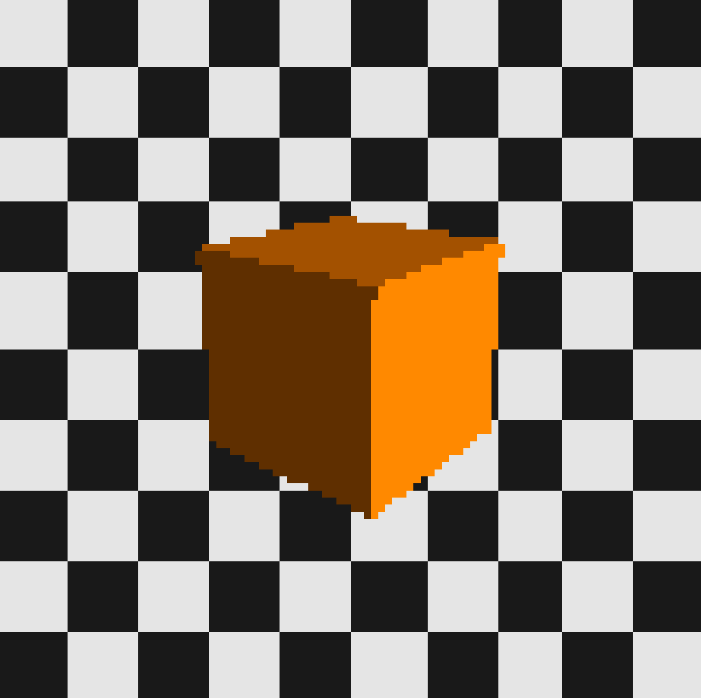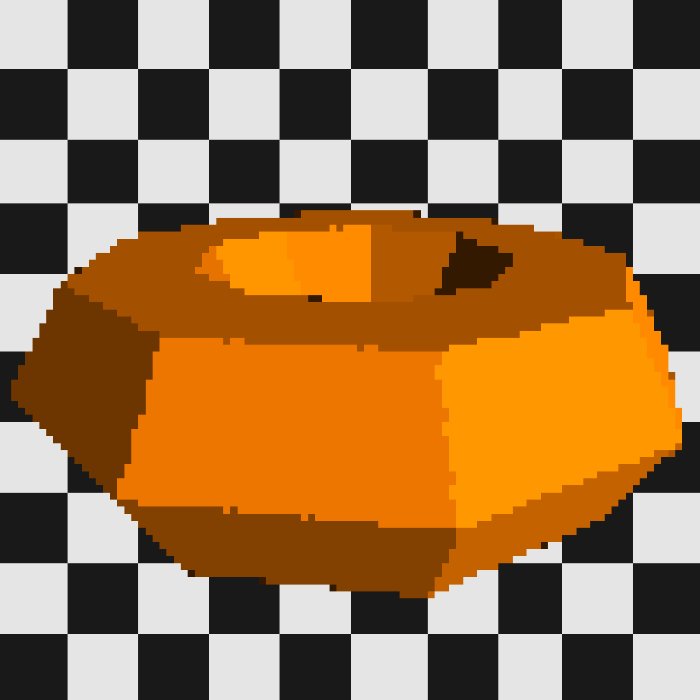Or, dare I try it, Suzanne?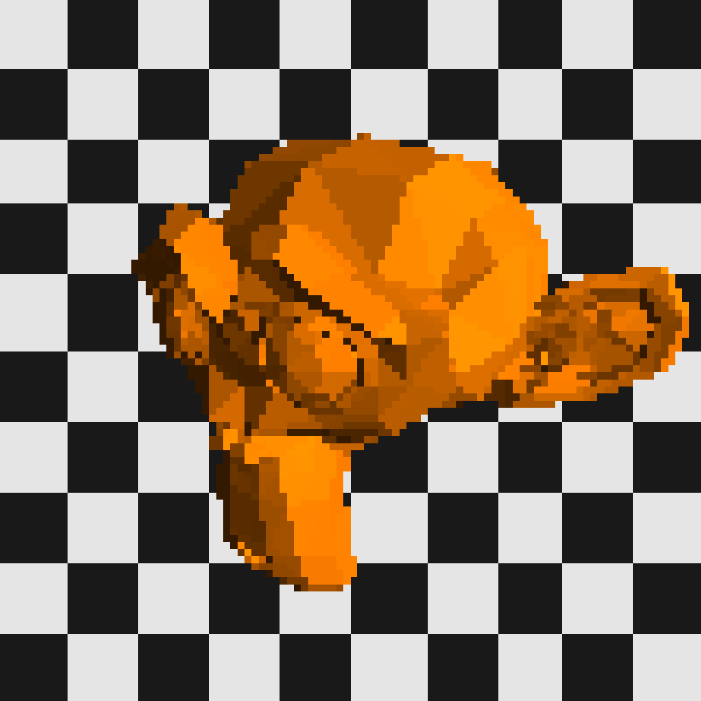## Conclusion

Different .obj files can now be traced by changing just one line and running the Lua script again, with no need for recompilation. This is very cool and is also a big part of why I want to do this in Lua - soon we will be needing to debug a large amount of graphics code, and graphics code are very, very hard to debug. However when the whole thing’s in Lua, we can iterate & debug extremely fast, by running the same patch of code over and over again, with little to no down time waiting for the project to recompile. We can also just change one line of code to see the change in render result. And on top of it all, it’s just very cool.

However, the limitation of Lua is starting to show. A simple 100x100 raytrace takes quite some time - the cube needs at least 10 seconds, the torus 60, and the monkey took like 90 seconds or so. With each new triangle the complexity to trace the scene will definitely explode, not to mention we didn’t even start solving the rendering equation yet. BVH and multithreading should be implemented sooner or later or the whole thing will become unusable.

There’s another problem in the intersect code presented above. This only works for flat-shaded meshes where each vertex of a face has the same normals - smooth shaded mesh’s normals will vary, making the above code janky. One way to solve this is to calculate real normals on the fly, or use another intersection algorithm. I guess I’ll see what I can do. So as always, stay tuned for more!

]]>
Writing a Pathtracer in Lua, Week 12023-08-12T20:00:30+08:002023-08-12T20:00:30+08:00https://blog.42yeah.is/rendering/2023/08/12/luaptHey guys! This week, it’s gonna be a little unusual. I plan to start a multi-week series to implement a Pathtracer in Lua. Well not everything is to be implemented in Lua, but most of the stuffs. Why Lua you ask? Because 1. Lua is a very flexible language and I can just hot-reload my Pathtracing code whenever I feel like, and 2. the result can be shown instantaneously. No more tedious waiting for the monstrously slow C++ codebase to compile. I know there’s Ninja and everything but Visual Studio really did a number on me. I hope you can go on this beautiful journey with me as well, random internet stranger!

## Project Structure

The project itself will be called LuaPT. The heavy-lifting and core code will be done in C++, while the actual pathtracing code will be written in Lua. In this case, we can hot-reload the pathtracer in real time when changes are made, making the whole process extremely quick.

But it’s kind of hard to distinguish between heavy-lifting and actual pathtracing. Model loading, image I/O and the like will obviously be a C++ task, but it’s not like pathtracing is a trivial task. Therefore, here are my plans so far:

• Model loading, image I/O, or just about every I/O related thing will be written in C++.
• Thread workers and management will be written in C++, but it will be Lua which gives them tasks.
• The pathtracer will be visualized using GLFW & ImGui (C++)
• BVH construction is somewhere in the middle and so both can take care of it (preferrably C++?).
• Ray sampling, Monte Carlo integration, etc. is done completely in Lua.

## Flowchart

Here’s a super simple flowchart demonstrating the workflow.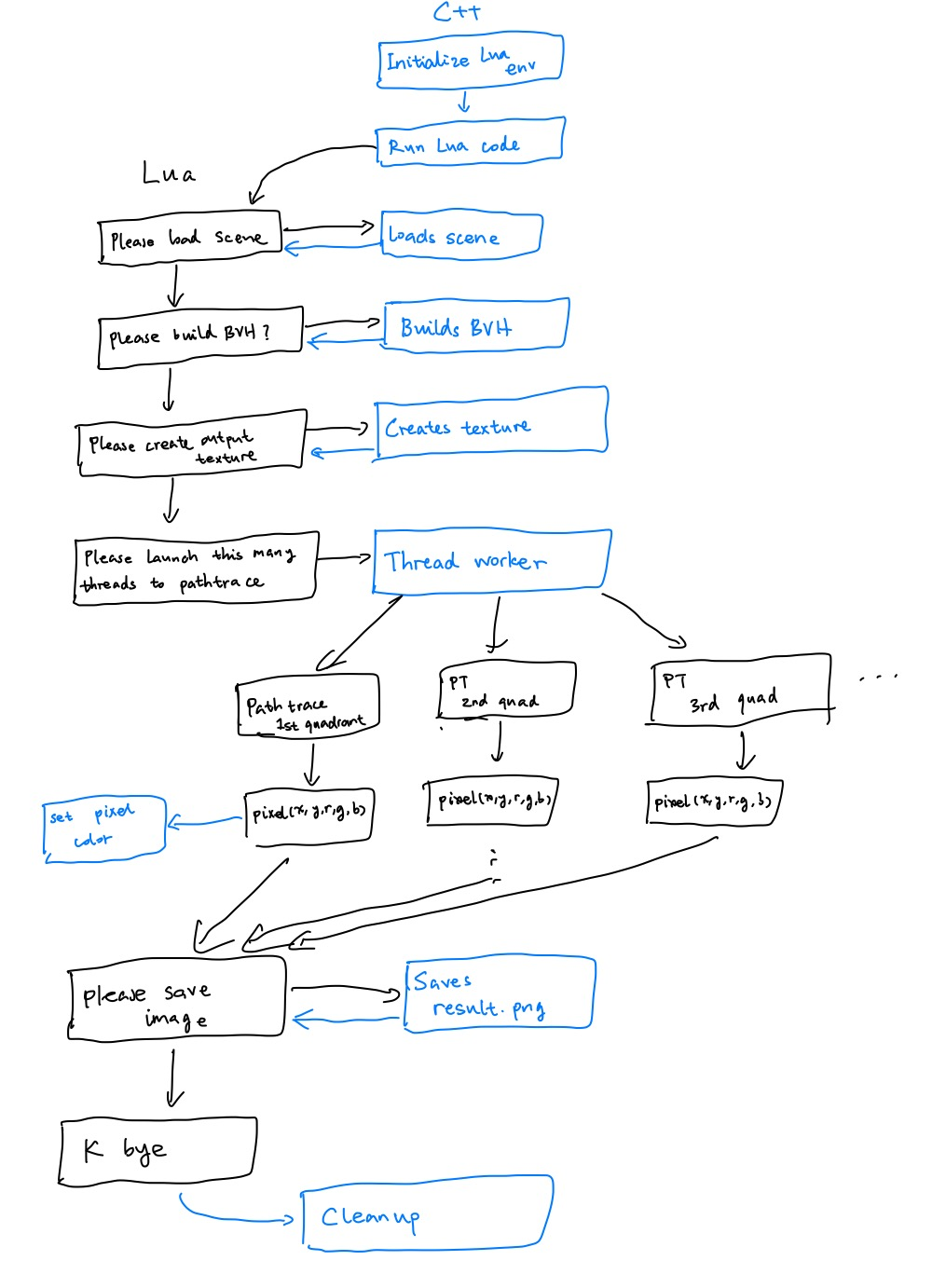The blue parts will be done in C++, and the black part in Lua. As we can see, Lua is politely asking C++ to do things most of the time. However, the actual pathtracing part is in Lua. That means we can either pass the whole scene into Lua, and implement the intersect function, or having Lua invoke the intersect function implemented in C++. You know, it looks almost like an engine. I guess I will know more as the design progresses.

## Image I/O

And now it’s time to get to work! We’ll be implementing the Image class first; after all, what’s a pathtracer’s worth if it cannot output images?

using CComp = unsigned char; // CComp for color component

struct RGB
{
CComp r;
CComp g;
CComp b;
};

class Image
{
public:
/**
* Default constructor
*/
Image();

unsigned int at(int x, int y) const;
void set_rgb(int x, int y, CComp r, CComp g, CComp b);
void set_rgb(int x, int y, const RGB &rgb);
RGB get_rgb(int x, int y) const;
bool save(const std::string &dest) const;

/**
* Copy constructor
*
* @param other The other image, to be copied from.
*/
Image(const Image& other);

/**
* Destructor
*/
~Image();

int id() const;

int w, h;

private:
std::unique_ptr<CComp[]> image;
int id_;
bool initialized;
};


The Image class holds a unique_ptr which will be freed upon going out of scope. In this way, we can manage the memory usage efficiently. The implementation involves using stb_image.h and stb_image_write.h of the stb library. We will only showcase some key functions below because dumping the whole source file in a blog post is a massive waste of space.

The image is initialized by setting the values of all of its channels to 0 (including alpha). Right now we only support RGBA image - even though we won’t be using the alpha channel anytime soon.

Image::Image(int w, int h, int ch) : w(w), h(h), image(nullptr), id_(::id++), initialized(false)
{
assert(ch == num_ch && "Unsupported: channels != 4");
image.reset(new CComp[w * h * num_ch]);
initialized = true;
for (int y = 0; y < h; y++)
{
for (int x = 0; x < w; x++)
{
image[at(x, y) + 0] = 0;
image[at(x, y) + 1] = 0;
image[at(x, y) + 2] = 0;
image[at(x, y) + 3] = 0;
}
}
}


The at function asserts that the sampling position is correct and calculates the proper offset. Only initialized images can be used.

unsigned int Image::at(int x, int y) const
{
assert(initialized && "Image is not initialized");

if (x < 0) { x = 0; }
if (y < 0) { y = 0; }
if (x >= w) { x = w - 1; }
if (y >= h) { y = h - 1; }
return (y * w + x) * num_ch;
}


## The Lua Environment

Now to set up the Lua environment. Other than the classic lua_State pointer, we will hold all Lua-related variables in the Lua class as well.

/**
* The Lua interface. Responsible for loading in scripts and executing them.
* The whole thing is designed to be a giant state machine, and therefore non-copyable.
*/
class Lua
{
public:
/**
* No copy constructor
*/
Lua(const Lua &another) = delete;

/**
* Destructor
*/
~Lua();

int execute(const std::string &buffer);
int execute_file(const std::string &file);

void report_error(const std::string &msg);
void register_funcs();

std::vector<std::string> err_log;

static std::shared_ptr<Lua> inst();

private:
/**
* Default constructor
* Thou shalt not call me directly, for I am a singleton
*/
Lua();

lua_State *l;
std::vector<std::shared_ptr<Image> > images;
};


The Lua class is a singleton and can only be accessed using inst(), in which it will be lazy-initialized (Lua() is private). It is capable of executing both lines of code and files. An error log is kept so that errors are easy to trace. Right now, when LuaPT launches, a CLI shows and accepts file names as input. Lua::inst()->execute_file() is then executed with errors logged into err_log. Then the CLI asks for another file name and executes that. Then it loops until EOF is reached.

In the Lua constructor, it will invoke register_funcs in which C++-Lua interoperability functions are registered, for example, creating/saving an image:

void Lua::register_funcs()
{
lua_register(l, "make_image", make_image);
lua_register(l, "set_pixel", set_pixel);
lua_register(l, "save_image", save_image);
lua_register(l, "free_image", free_image);
}


## Lua Wrappers & GC

As Lua can’t just invoke constructors in C++, we need to implement extra functions so that images can be managed in Lua. We achieve this by having an Image vector in the Lua class, so that make_image can just be inserting a new image into said vector and returning the index. We can then manipulate the image in Lua using the handle we got. When deleting the image, we call free_image in Lua so that it is removed from the vector. The smart pointer will now have 0 reference and free itself.

But why not embrace Lua’s intrinsic GC as well? Lua can garbage collect things when they are left alone after a while. This is quite useful when, for example, the codebase in Lua becomes complicated down the line and resource management becomes difficult. To make things easier for us, we can wrap the functions up so they become pseudo classes (again), but this time, it’s in Lua.

Image = {
handle = 0,
w = 0,
h = 0,
__gc = function()
if w ~= 0 and h ~= 0 then
free_image(self.handle)
end
end
}

function Image:new(w, h)
local ret = {}
setmetatable(ret, self)
self.__index = self
ret.handle = make_image(w, h)
ret.w = w
ret.h = h
return ret
end

function Image:save(name)
save_image(self.handle, name)
end

function Image:pixel(x, y, r, g, b)
set_pixel(self.handle, x, y, clamp(r, 0, 1), clamp(g, 0, 1), clamp(b, 0, 1))
end

function Image:pixel_vec4(x, y, vec4)
set_pixel(self.handle, x, y, clamp(vec4.x, 0, 1), clamp(vec4.y, 0, 1), clamp(vec4.z, 0, 1))
end


So to recap, we implemented the image I/O in C++, as classes, then wrapped them up as functions. Then in Lua, we wrapped the functions up (again) and they become classes.

## Current Result

That’s all we’ve implemented so far. A way for Lua to save/write the image. Concurrency, model loading, etc. is still further down the line.

But we can still have some impressive result. For example, feast your eyes on THIS! After implementing a small math library in Lua later, we have raymarched a ball using the very thing we just made.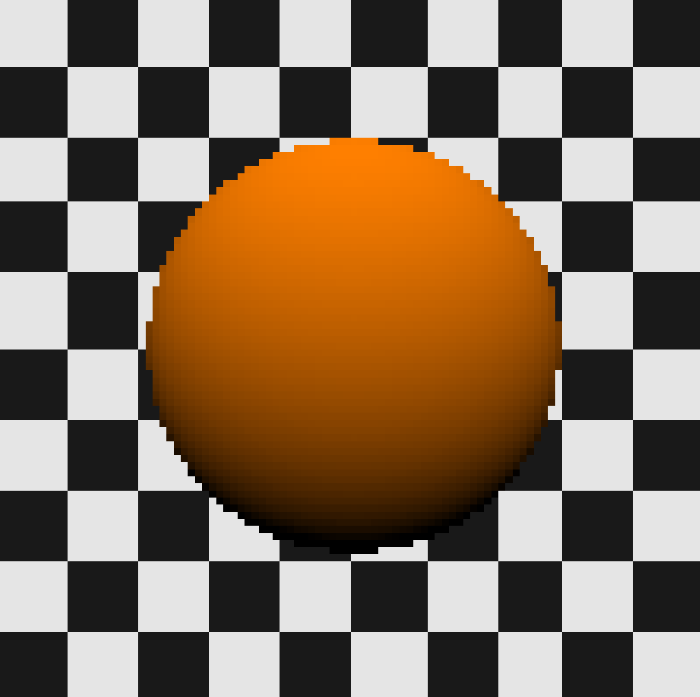require "lib/image"
require "math"
local pprint = require "lib/pprint"

local im = Image:new(100, 100)

ro = Vec4:new(0, 0, -2, 1)
center = Vec4:new(0, 0, 0, 1)
front = center:subtr(ro):nor3() -- most of the time we won't be needing the 4th component
right = front:cross(Vec4:new(0, 1, 0, 1)):nor3()
up = right:cross(front):nor3()

t = 0.01

for i = 1, 200 do

-- try to sample a sphere located at center with r=0.5
r = 1.0
d = p:len3() - r
if d < 0.01 then
-- a sphere at the center has a special case normal where the position being exactly the opposite of the normal direction
nor = p:nor3():scl(-1)
nor.y = -nor.y
brightness = math.max(0.0, nor:dot3(Vec4:new(0, 1, 1, 1):nor3()))
return Vec4:new(1.0, 0.5, 0.0, 1.0):scl(brightness)
end
t = t + d
if t > 20 then
break
end
end

-- color background
uu = math.floor(u * 5)
vv = math.floor(v * 5)
if (uu + vv) % 2 == 0 then
return Vec4:new(0.1, 0.1, 0.1, 1)
else
return Vec4:new(0.9, 0.9, 0.9, 1)
end

end

for y = 1, 100 do
for x = 1, 100 do
u = (x - 1) / 100.0 * 2.0 - 1.0
v = (y - 1) / 100.0 * 2.0 - 1.0
res = shade(u, v, x - 1, y - 1)
im:pixel_vec4(x - 1, y - 1, res)
end
end

im:save("rm.png")


I will smooth the project around the edges and implement it bit by bit in the coming weeks. By posting it onto my blog, it now has automatically become a huge sunken cost and now I can’t abandon it anymore (?). So stay tuned for more!

]]>
A Two-Person Method to Simulate Die Rolls2023-08-05T22:16:30+08:002023-08-05T22:16:30+08:00https://blog.42yeah.is/algorithm/2023/08/05/two-person-dieI’ve traveled to Xi’an to play with my friend, Zambon21. There are some boring time which must be passed, and so we tried to pass them using brain-procedurally generated simplified D&D-like campaigns. As all D&D campaigns requires dice, ours is no exception. But by then we were presented with no other tools except our mortal bodies (because both of our phone’s batteries had run out), and mortal bodies are quite bad at generating random numbers. Inspiration struck when we wandered straight into a tourist trap. Exploiting the polar coordinate, we thought up a neat little trick to generate random numbers, and simplified it so that it can simulate a randomly generated die roll. Along with the method, we bundled a fun little game that can be played with no other tools other than the fake dice we invented above. After all, who doesn’t love random numbers?

## Methodology

We will present the random number generation method below. The method is capable of generating numbers between $$[0, 1)$$ and requires the participation of two parties, and two positions situated on the unit circle of the polar coordinate system to work.

1. A number ($$\theta_1$$) is proposed by A.
2. Another number ($$\theta_2$$) is proposed by B.
3. The angular difference is evaluated ($$\delta$$).
4. The angular difference is normalized: $$\delta = \frac{\delta}{\pi}$$.

If A and B are biased and are not capable of generating real random numbers on their own, but they have a conflict of interest, this will cause both A and B to try to maximize or minimize the angular difference, and therefore, producing a semi-random result. Cool!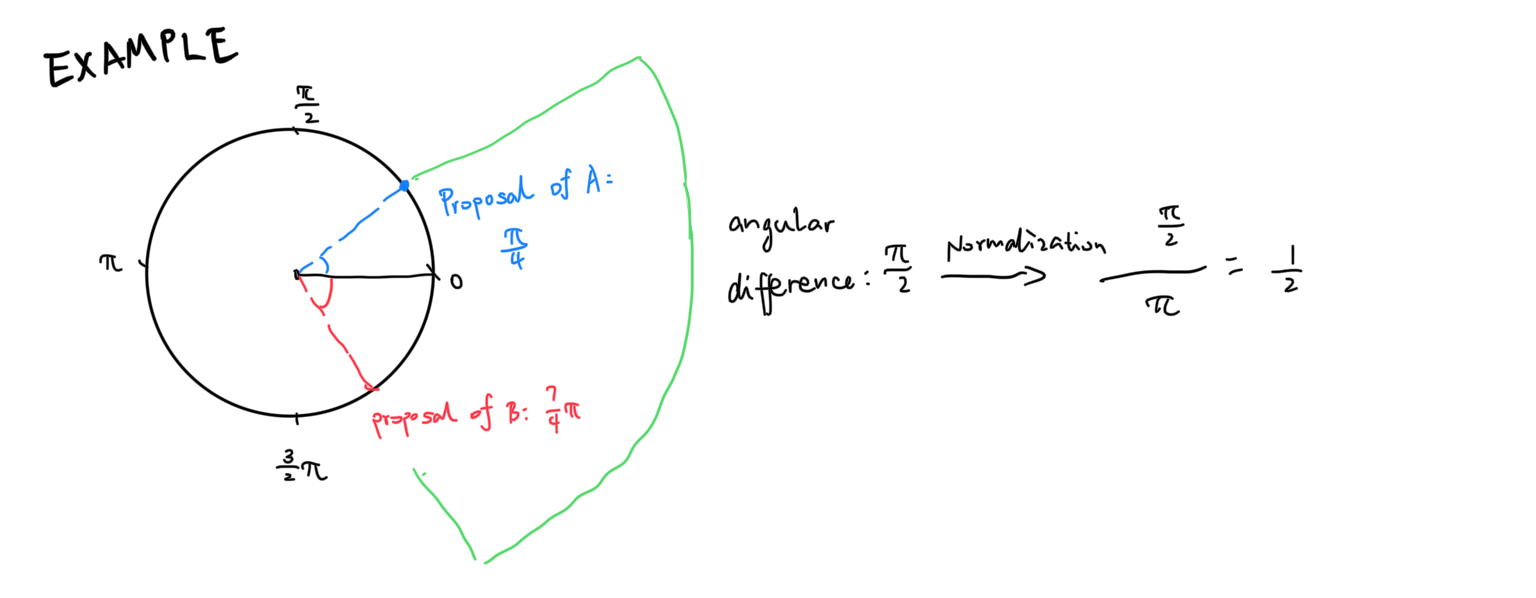If the difference $$\delta$$ is bigger than $$\pi$$, we invert it using $$\delta \rightarrow \delta - \pi$$, so the RNG is always capped between 0 to 1.

## Discretization

Obviously humans are very inept at generating continuous fake random numbers, and the method above is 1. not very intuitive and 2. not a die and therefore not usable in a vastly simplified D&D campaign. We simplified the algorithm so that both challenges can be solved.

First, we rotate the above polar coordinate by 90deg so that 0 is on the top. Then, we flip it along the y axis so it becomes clockwise. Basically, we turn it into a literal clock. We mark the circle with clock numbers as well.Two parties come up with a number ranged between $$[0, 12)$$ simultaneously to avoid the information gap. We then measure their angular difference. For example, A comes up with 2 and B comes up with 10. The angular difference will be 4 in this case. The fake die roll result is then calculated using the angular difference.

---------------------------------
|  Angular Distance | Die roll  |
---------------------------------
|  0, 6             |    6      |
|  1                |    1      |
|  2                |    2      |
|  3                |    3      |
|  4                |    4      |
|  5                |    5      |
---------------------------------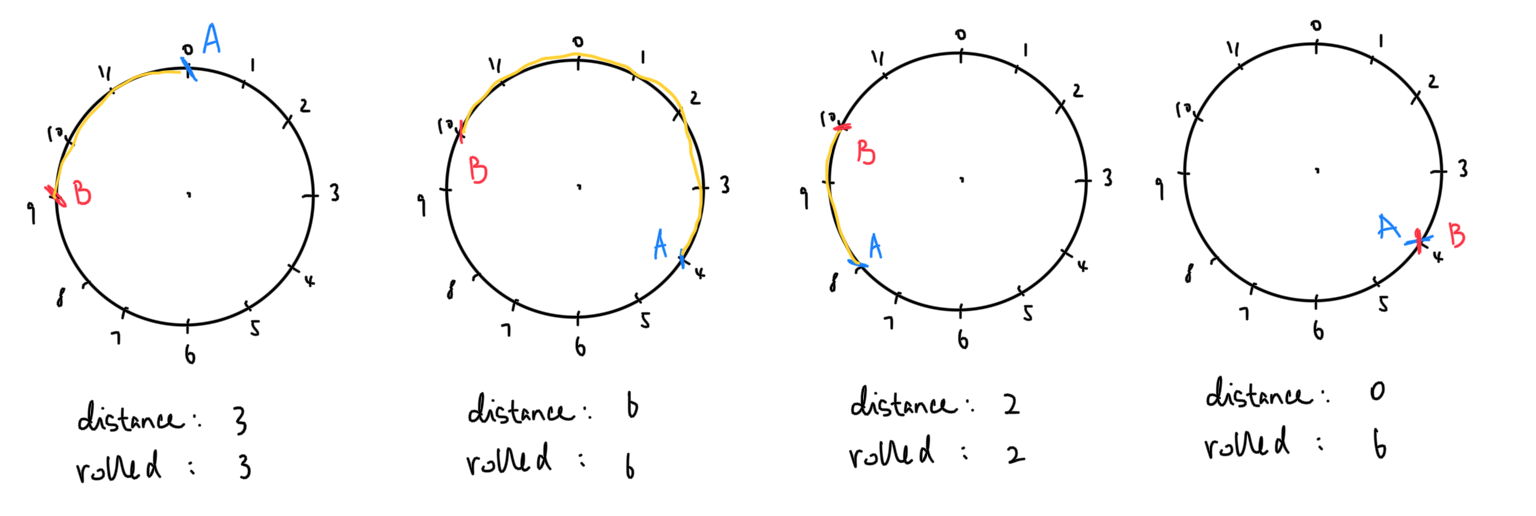We can prove that in an ideal situation, the die roll will be fair. Assuming both parties can come up with unbiased random numbers ranging from $$[0, 12)$$, we can always rewrite the clock numbers so that A is always situated at 0. This means $$P_A(0) = 1$$, and the probability for the resulting distance now entirely depend on the probability of where B lands. This is due to something to the effect of distance is rotational invariant.---------------------------------------------
| Distance    | Landing point | Probability |
---------------------------------------------
|   0         |       0       |   1/12      |
|   1         |     1, 11     |   1/6       |
|   2         |     2, 10     |   1/6       |
|   3         |     3, 9      |   1/6       |
|   4         |     4, 8      |   1/6       |
|   5         |     5, 7      |   1/6       |
|   6         |       6       |   1/12      |
---------------------------------------------


We don’t have to lock A to 0 - any party can become the anchor point. Due to the symmetry, it doesn’t really matter. The result will be the same. Distance 1-5 conveniently have the same probability with the probability of a die roll - $$\frac{1}{6}$$; 0 and 6 not so much, with a probability of $$\frac{1}{12}$$. As a result, we combine them together to mark the die roll of 6.

## Experiment

We have written a little experiment in Lua to verify the things we said above.

diecount = {}

function propose()
return math.floor(math.random() * 12)
end

function wrap(x)
if x < 0 then
return x + 12
end
if x >= 12 then
return x - 12
end
return x
end

function pdistance(a, b)
local lut = {
0, 1, 2, 3, 4, 5, 6, 5, 4, 3, 2, 1
}
return lut[wrap(b - a) + 1]
end

function roll()
a = propose()
b = propose()
pt = pdistance(a, b)
if pt == 0 then
pt = 6
end
diecount[pt] = diecount[pt] + 1
end


By offsetting B with -A, we can evaluate the angular distance using a lookup table, which saves up to 2 ifs.

Here’s the statistics after executing the roll() function above for 1,000,000 times. A fair die should have equal probability for each side, that is, 1/6, or 16.66666…%:

--------------------------------
| Roll   | Count  |    %       |
--------------------------------
|  1     | 166390 |  16.639%   |
|  2     | 167068 |  16.7068%  |
|  3     | 166697 |  16.6697%  |
|  4     | 166385 |  16.6385%  |
|  5     | 166622 |  16.6622%  |
|  6     | 166838 |  16.6838%  |
--------------------------------


## Verbal Tennis

Besides verbal D&D, me and my friend had come up with a little game based on the die roll: verbal tennis. In verbal tennis, each player starts with 3 points of stamina. When player 1 serves the ball, they can choose whether to spend stamina or not. Used bar of stamina will grow back after 3 turns. Player 1 and player 2 then says a random number together at the same time to generate a die number (a). Player 2 can only successfully return the ball if they get (a + stamina used) or above.

Just like player 1 can punish the die count by spending stamina, player 2 can award the die count by spending their stamina as well. Player 2 additionally have the option to spend stamina to punish player 1’s roll, should the ball be successfully returned. The game ends when one player wins using whatever tennis rules you guys choose to use. Here’s a very immaturely drawn comic strip demonstrating the game procedure.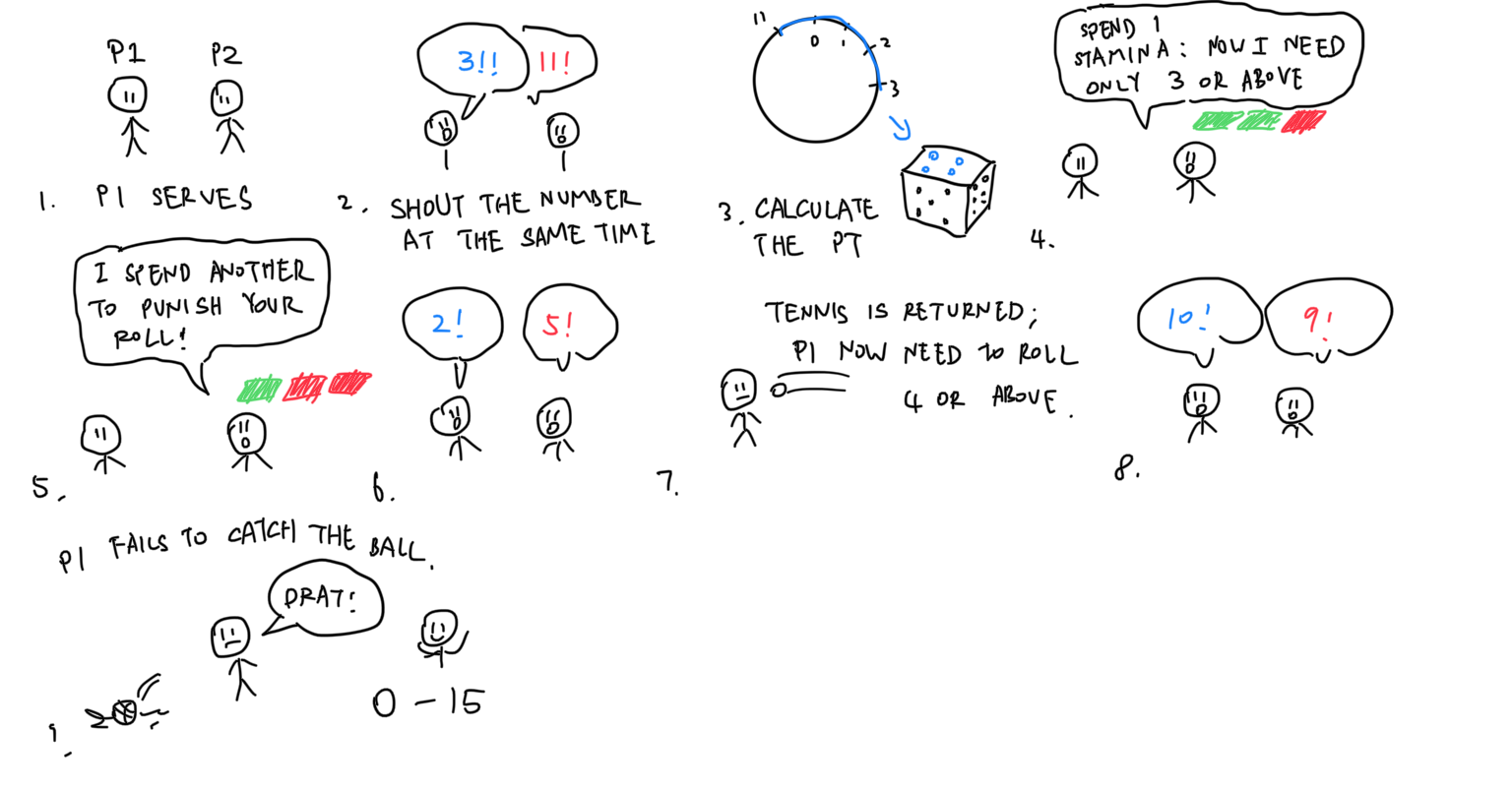## Conclusion

That’s it! One neat little trick we’ve discovered to roll a die, without a die. The method is not without its flaw though. To at least make it fair, both participants will need to have opposite targets or interests, otherwise the roll result might be rigged. So one side must be actively trying to achieve the minimal roll, while the other side trying to maximize it. And of course, when there is an actual die in your possession, or you have things like Sophie’s Dice, this method becomes useless. The third downside is that the discretized method can only roll a d6; there might simply be too many numbers to track if you want to roll a d20. Finally, although this is not what this blog should be posting, conventionally speaking, I hope you guys had fun reading it. And of course, feel free to use it next time in your oversimplified D&D campaign!

]]>